# NYT and WaPo Data Visualizations on Carbon Emissions Recreated in R

After President Trump declared that the U.S. was pulling out of the Paris agreement on climate change, the New York Times and Washington Post published a couple of stories. In these stories, they included a few charts. They caught my eye and I wanted to see whether I could tell similar stories and draw some more conclusions using the data and R. In this post, similar to my previous posts, you will see my efforts to create the NYT and Washington Post data visualizations recreated in R.

### Reasons these graphics are good

• Color: both the NYT and WaPo chose simple color schemes. They didn’t use the colors to “prettify”, but actually to distinguish countries. The NYT also followed the same color scheme throughout the article.
• Chart Type: all the graphics have the right chart types. Area charts, which really are extensions of line charts, are good choices to show growth over time. I personally prefer line charts, because they gave similar information without cluttering the plot. Horizontal-bar charts are also good choices for showing a measure across categories. Turning the axis helps read the category labels with ease. Although the circle charts, a.k.a bubble charts, look good, they take a lot of space to inform the readers.

# Experts Advice on Colors and Graphics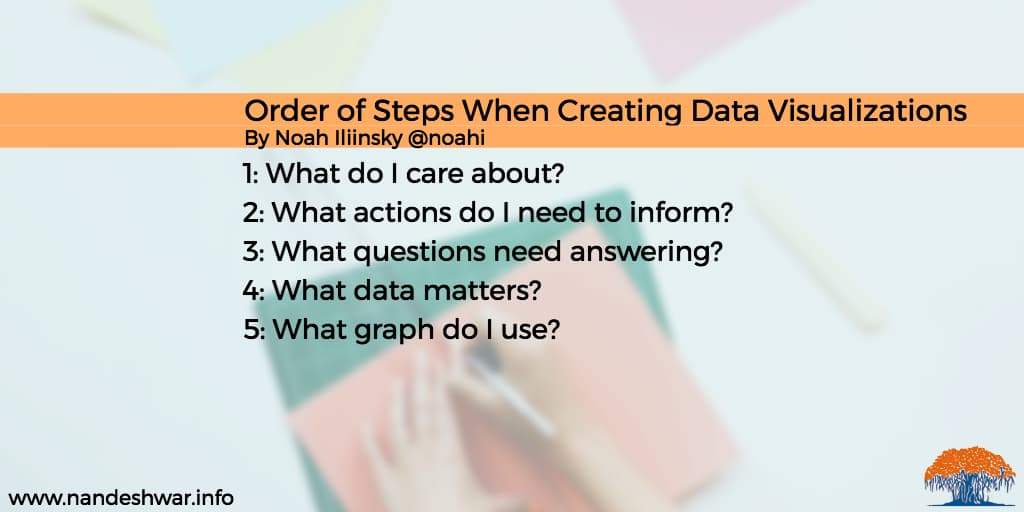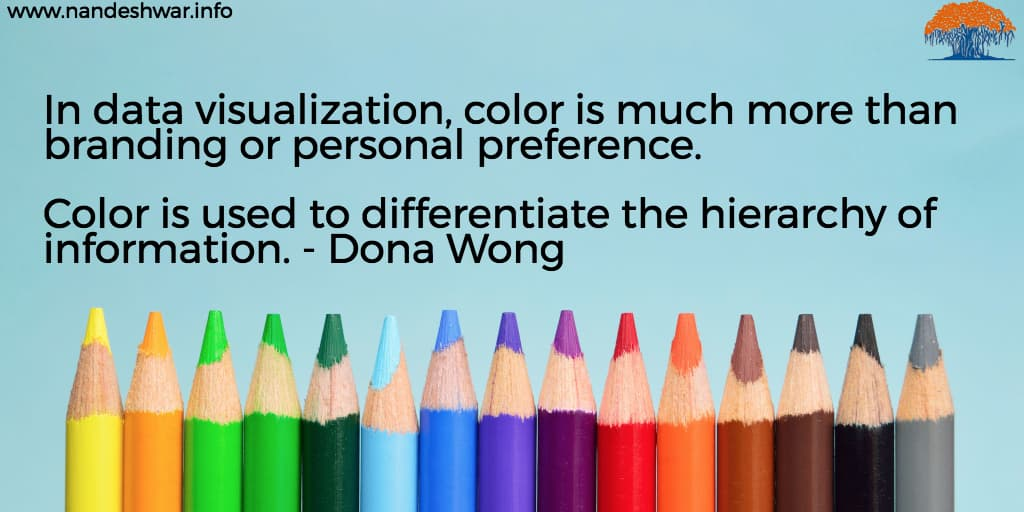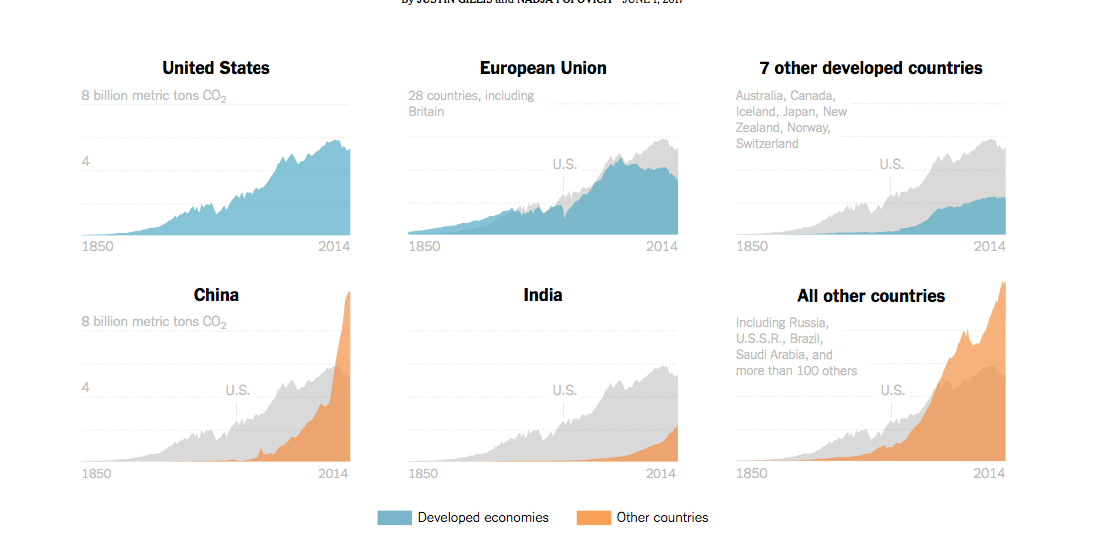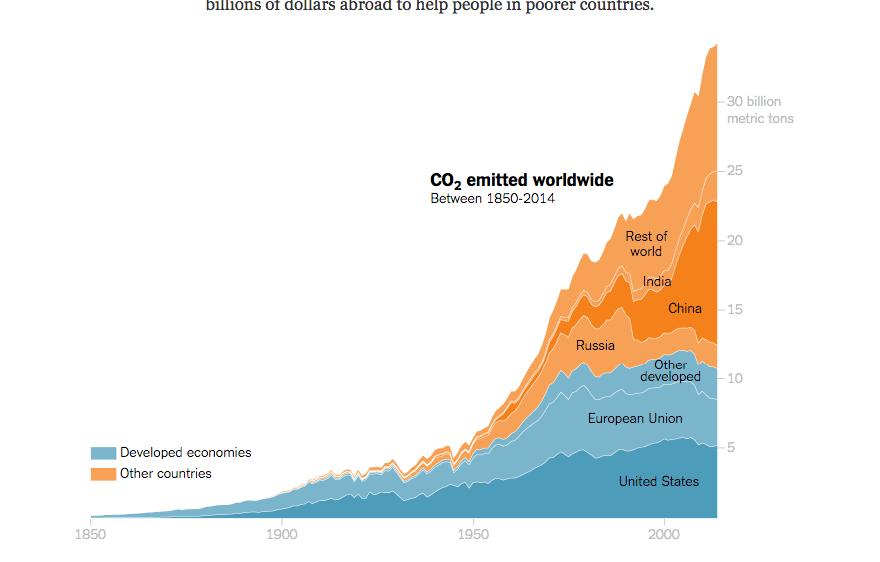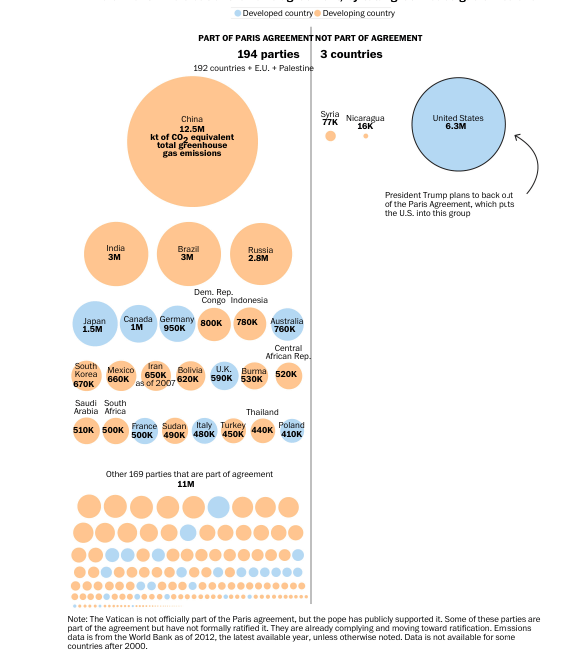# NYT Graphics

Let’s create the NYT graphics first.

```library(stringr) library(dplyr) library(ggplot2) library(scales) library(ggmap) library(readr) library(maps) library(tidyr) library(rvest)```

Get the data from the source. Here, I am using the carbon emissions data by each nation. The missing values are denoted by a period and the first four lines have comments. I specified those as additional arguments for the `read_csv` function.

`emissions_by_countries <- read_csv("http://cdiac.ornl.gov/ftp/ndp030/CSV-FILES/nation.1751_2014.csv", col_names = c("country", "year", "total_emissions", "gas_fuel_emissions", "liquid_fuel_emissions", "solid_fuel_emissions", "gas_flaring_emissions", "cement_prod_emissions", "per_capita_emission_rate", "bunker_fuel_emissions"), na = ".", skip = 4)`

This data looks like this:

`glimpse(emissions_by_countries)`
``````## Observations: 17,232
## Variables: 10
## \$ country                   "AFGHANISTAN", "AFGHANISTAN", "AFGHAN...
## \$ year                      1949, 1950, 1951, 1952, 1953, 1954, 1...
## \$ total_emissions           4, 23, 25, 25, 29, 29, 42, 50, 80, 90...
## \$ gas_fuel_emissions        4, 6, 7, 9, 10, 12, 17, 17, 21, 25, 3...
## \$ liquid_fuel_emissions     0, 18, 18, 17, 18, 18, 25, 33, 59, 65...
## \$ solid_fuel_emissions      0, 0, 0, 0, 0, 0, 0, 0, 0, 0, 0, 0, 0...
## \$ gas_flaring_emissions     0, 0, 0, 0, 0, 0, 0, 0, 0, 0, 5, 5, 6...
## \$ cement_prod_emissions     NA, 0, 0, 0, 0, 0, 0, 0, 0, 0, 0, 0, ...
## \$ per_capita_emission_rate  NA, 0.00, 0.00, 0.00, 0.00, 0.00, 0.0...
## \$ bunker_fuel_emissions     0, 0, 0, 0, 0, 0, 0, 0, 0, 0, 0, 0, 0...``````

Next, let’s do some clean-up and add some calculations. As per the data file, to get the CO2 emissions, we need to multiply the carbon emissions by a constant of 3.667, because one ton of carbon equals 3.667 tons of carbon dioxide gas.

```emissions_by_countries <- mutate(emissions_by_countries, country = str_to_title(country), co2_total_emissions = total_emissions * 3.667, co2_per_capita_emission_rate = per_capita_emission_rate * 3.667)```

Now the data looks like this:

`glimpse(emissions_by_countries)`
``````## Observations: 17,232
## Variables: 12
## \$ country                       "Afghanistan", "Afghanistan", "Af...
## \$ year                          1949, 1950, 1951, 1952, 1953, 195...
## \$ total_emissions               4, 23, 25, 25, 29, 29, 42, 50, 80...
## \$ gas_fuel_emissions            4, 6, 7, 9, 10, 12, 17, 17, 21, 2...
## \$ liquid_fuel_emissions         0, 18, 18, 17, 18, 18, 25, 33, 59...
## \$ solid_fuel_emissions          0, 0, 0, 0, 0, 0, 0, 0, 0, 0, 0, ...
## \$ gas_flaring_emissions         0, 0, 0, 0, 0, 0, 0, 0, 0, 0, 5, ...
## \$ cement_prod_emissions         NA, 0, 0, 0, 0, 0, 0, 0, 0, 0, 0,...
## \$ per_capita_emission_rate      NA, 0.00, 0.00, 0.00, 0.00, 0.00,...
## \$ bunker_fuel_emissions         0, 0, 0, 0, 0, 0, 0, 0, 0, 0, 0, ...
## \$ co2_total_emissions           14.668, 84.341, 91.675, 91.675, 1...
## \$ co2_per_capita_emission_rate  NA, 0.00000, 0.00000, 0.00000, 0....``````

## Get EU Countries

These charts use a group for the European Union. So, let’s get those countries from the EU’s site.

```eu_countries <- read_html("http://europa.eu/european-union/about-eu/countries_en") %>% html_node(xpath = '//*[@id="year-entry2"]/table') %>% html_table() %>% `names<-`(c("x1", "x2")) %>% gather(value = countries) %>% select(-key) %>% mutate(EU = 'EU')```

Line by line explanation of what’s happening in this code:

• download the whole page using `read_html`
• using Chrome’s `Inspect` and `Copy Xpath` select the table we need
• convert the html to a data frame
• since both columns has the same heading, give them some dummy names. `dplyr` doesn’t like duplicate column names
• fold both the columns into one using `gather` function from the package `tidyr`
• remove the extra column
• add a field to denote the EU

This data looks like this:

`glimpse(eu_countries)`
``````## Observations: 28
## Variables: 2
## \$ countries  "Austria", "Belgium", "Bulgaria", "Croatia", "Cyprus...
## \$ EU         "EU", "EU", "EU", "EU", "EU", "EU", "EU", "EU", "EU"...``````

Let’s make sure that all the EU countries exist in the carbon emissions data set.

`filter(eu_countries, !(countries %in% emissions_by_countries\$country)) %>% select(countries)`
``````##   countries
## 1    France
## 2     Italy``````

Since France and Italy has some additional text, let’s change those:

```emissions_by_countries <- mutate(emissions_by_countries, country = ifelse(country == 'France (Including Monaco)', 'France', ifelse(country == 'Italy (Including San Marino)', 'Italy', ifelse(country %in% c('Ussr', 'Russian Federation'), 'Russia', ifelse(country == 'China (Mainland)', 'China', country)))))```

Let’s now merge the EU data frame with the emissions data frame and add the EU column:

```emissions_by_countries <- left_join(emissions_by_countries, eu_countries, by = c("country" = "countries")) %>% mutate(EU = ifelse(is.na(EU), 'Non-EU', EU))```

This data frame looks like this:

`glimpse(emissions_by_countries)`
``````## Observations: 17,232
## Variables: 13
## \$ country                       "Afghanistan", "Afghanistan", "Af...
## \$ year                          1949, 1950, 1951, 1952, 1953, 195...
## \$ total_emissions               4, 23, 25, 25, 29, 29, 42, 50, 80...
## \$ gas_fuel_emissions            4, 6, 7, 9, 10, 12, 17, 17, 21, 2...
## \$ liquid_fuel_emissions         0, 18, 18, 17, 18, 18, 25, 33, 59...
## \$ solid_fuel_emissions          0, 0, 0, 0, 0, 0, 0, 0, 0, 0, 0, ...
## \$ gas_flaring_emissions         0, 0, 0, 0, 0, 0, 0, 0, 0, 0, 5, ...
## \$ cement_prod_emissions         NA, 0, 0, 0, 0, 0, 0, 0, 0, 0, 0,...
## \$ per_capita_emission_rate      NA, 0.00, 0.00, 0.00, 0.00, 0.00,...
## \$ bunker_fuel_emissions         0, 0, 0, 0, 0, 0, 0, 0, 0, 0, 0, ...
## \$ co2_total_emissions           14.668, 84.341, 91.675, 91.675, 1...
## \$ co2_per_capita_emission_rate  NA, 0.00000, 0.00000, 0.00000, 0....
## \$ EU                            "Non-EU", "Non-EU", "Non-EU", "No...``````

Let’s also add the economy type column:

```emissions_by_countries <- mutate(emissions_by_countries, economy_type = ifelse(country %in% c('Australia', 'Canada', 'Japan', 'New Zealand', 'Iceland', 'Norway', 'Switzerland', 'United States Of America') | EU == 'EU', 'Developed economies', 'Other countries'), chart_groups = ifelse(country %in% c('Australia', 'Canada', 'Japan', 'New Zealand', 'Iceland', 'Norway', 'Switzerland'), 'Other developed', ifelse(country == 'United States Of America', 'United States', ifelse(country == 'China', 'China', ifelse(country == 'India', 'India', ifelse(EU == 'EU', 'European Union', 'Rest of world'))))))```

This data now looks like this:

`glimpse(emissions_by_countries)`
``````## Observations: 17,232
## Variables: 15
## \$ country                       "Afghanistan", "Afghanistan", "Af...
## \$ year                          1949, 1950, 1951, 1952, 1953, 195...
## \$ total_emissions               4, 23, 25, 25, 29, 29, 42, 50, 80...
## \$ gas_fuel_emissions            4, 6, 7, 9, 10, 12, 17, 17, 21, 2...
## \$ liquid_fuel_emissions         0, 18, 18, 17, 18, 18, 25, 33, 59...
## \$ solid_fuel_emissions          0, 0, 0, 0, 0, 0, 0, 0, 0, 0, 0, ...
## \$ gas_flaring_emissions         0, 0, 0, 0, 0, 0, 0, 0, 0, 0, 5, ...
## \$ cement_prod_emissions         NA, 0, 0, 0, 0, 0, 0, 0, 0, 0, 0,...
## \$ per_capita_emission_rate      NA, 0.00, 0.00, 0.00, 0.00, 0.00,...
## \$ bunker_fuel_emissions         0, 0, 0, 0, 0, 0, 0, 0, 0, 0, 0, ...
## \$ co2_total_emissions           14.668, 84.341, 91.675, 91.675, 1...
## \$ co2_per_capita_emission_rate  NA, 0.00000, 0.00000, 0.00000, 0....
## \$ EU                            "Non-EU", "Non-EU", "Non-EU", "No...
## \$ economy_type                  "Other countries", "Other countri...
## \$ chart_groups                  "Rest of world", "Rest of world",...``````

## Let’s start plotting

Now that we have our data together, let the fun begin.

First, let’s define a formatter to convert the emissions to billions of units.

```formatter_billions <- function(x){ x/10^9 }```

Next, let’s total the emissions by the group types as used in the NYT graphic:

```by_chart_groups <- group_by(emissions_by_countries, year, chart_groups, economy_type) %>% summarize(total_emissions = sum(co2_total_emissions*10^3, na.rm = TRUE)) %>% ungroup() %>% mutate(chart_groups = factor(chart_groups, levels = c('United States', 'European Union', 'Other developed', 'China', 'India', 'Rest of world')))```
`glimpse(by_chart_groups)`
``````## Observations: 1,132
## Variables: 4
## \$ year             1751, 1752, 1753, 1754, 1755, 1756, 1757, 1758...
## \$ chart_groups     European Union, European Union, European Unio...
## \$ economy_type     "Developed economies", "Developed economies", ...
## \$ total_emissions  9358184, 9361851, 9361851, 9365518, 9369185, 1...``````

Since we need the U.S. data along with every other group, we need to combine the U.S. data with each of the already grouped data.

```us_only <- filter(by_chart_groups, chart_groups == 'United States')   us_repeated <- us_only %>% slice(rep(1:n(), each = 6)) %>% mutate(chart_groups = rep(c('China', 'India', 'European Union', 'Other developed', 'Rest of world', 'United States'), nrow(us_only)), economy_type = NA) %>% mutate(chart_groups = factor(chart_groups, levels = c('United States', 'European Union', 'Other developed', 'China', 'India', 'Rest of world')))```

Now, the data frame `us_repeated` has every group’s data, including the U.S., and also has US’s data combined with it.

`glimpse(us_repeated)`
``````## Observations: 1,290
## Variables: 4
## \$ year             1800, 1800, 1800, 1800, 1800, 1800, 1801, 1801...
## \$ chart_groups     China, India, European Union, Other developed...
## \$ economy_type     NA, NA, NA, NA, NA, NA, NA, NA, NA, NA, NA, NA...
## \$ total_emissions  253023, 253023, 253023, 253023, 253023, 253023...``````

Next, let’s prepare the annotations on the graph:

```annotations_txt <- data.frame(chart_groups = c('United States', 'European Union', 'Other developed', 'China', 'India', 'Rest of world'), x = 1850, y = 8*10^9, txt = c('billion metric tons of CO2', '28 countries including\nBritain', 'Australia, Canada,\nIceland, Japan, New\nZealand, Norway,\nSwitzerland', 'billion metric tons of CO2', NA, 'Including Russia,\nU.S.S.R., Brazil,\nSaudi Arabia, and\nmore than 100 others'))```
`glimpse(annotations_txt)`
``````## Observations: 6
## Variables: 4
## \$ chart_groups  United States, European Union, Other developed, ...
## \$ x             1850, 1850, 1850, 1850, 1850, 1850
## \$ y             8e+09, 8e+09, 8e+09, 8e+09, 8e+09, 8e+09
## \$ txt           billion metric tons of CO2, 28 countries includi...``````

Build the color palette. I used imagecolorpicker to get the colors used in the NYT graphic.

`econ_type_colors <- c("Developed economies" = "#86C3D6", "Other countries" = "#F4AE7B")`

Now, let’s build the area graphs:

`g <- ggplot(us_repeated, aes(x = year, y = total_emissions)) + geom_area(fill = "gray85", alpha = 0.8) + facet_wrap(~chart_groups, nrow = 2)`

This command is straightforward: we are using the `year` column for the x-axis and the `total_emissions` column for the y-axis. We are then creating `facets` or `panels` a.k.a https://en.wikipedia.org/wiki/Small_multiple for the different chart groups.

We get this: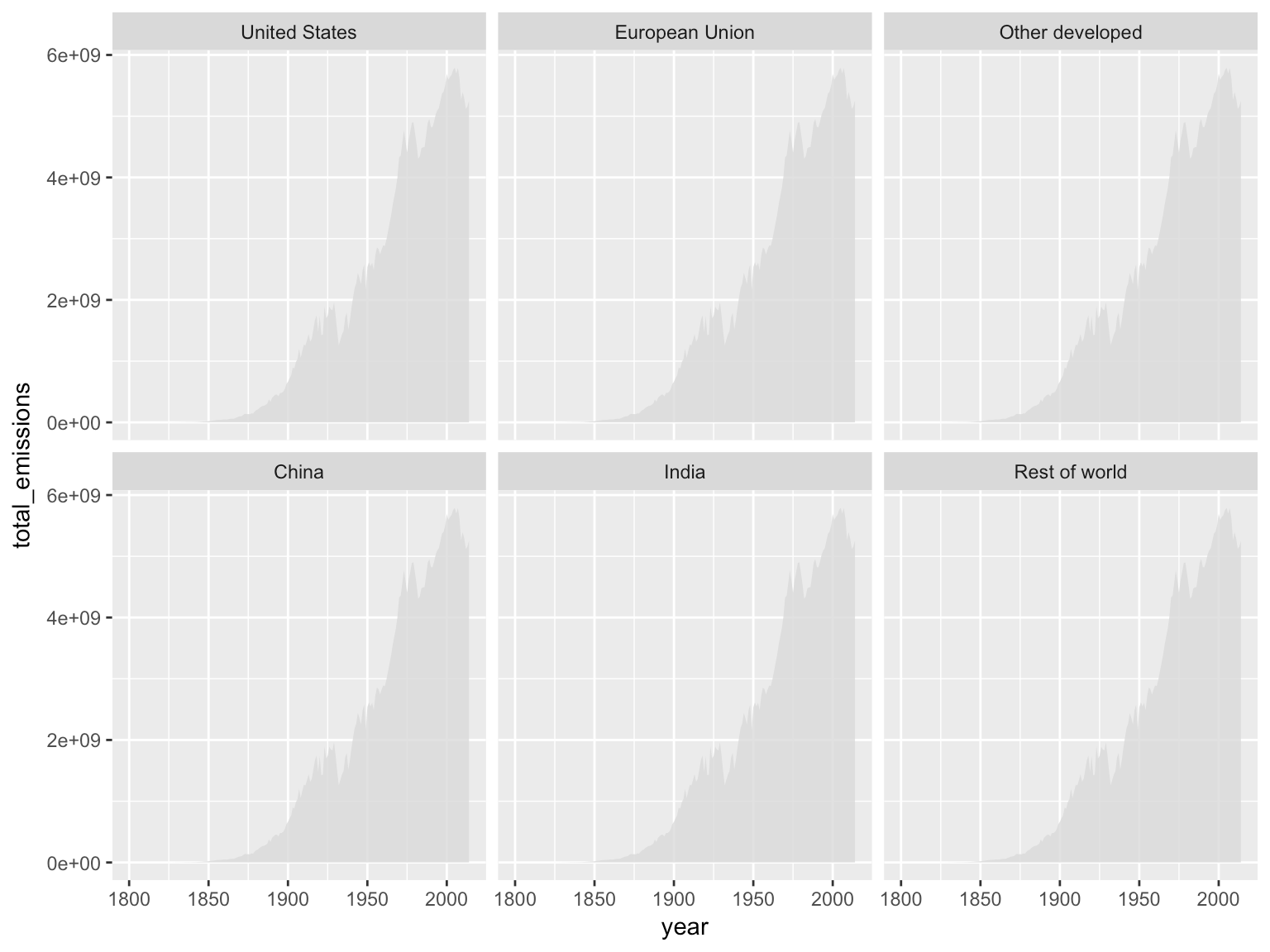You see all of the US data plotted neatly in its gray beauty! And, your reaction may be like:Now, let’s make you feel better and make some changes to the axes:

`g <- g + scale_x_continuous(limits = c(1850, 2014), breaks = c(1850, 2014)) + scale_y_continuous(labels = formatter_billions, limits = c(0,8*10^9), breaks = c(0, 4, 8)*10^9, minor_breaks = c(2, 6)*10^9)`

We are limiting the x-axis to two values: 1850 and 2014. We are formatting the y-axis to show billions as a unit and to have the grid lines at 0, 4, and 8 billion.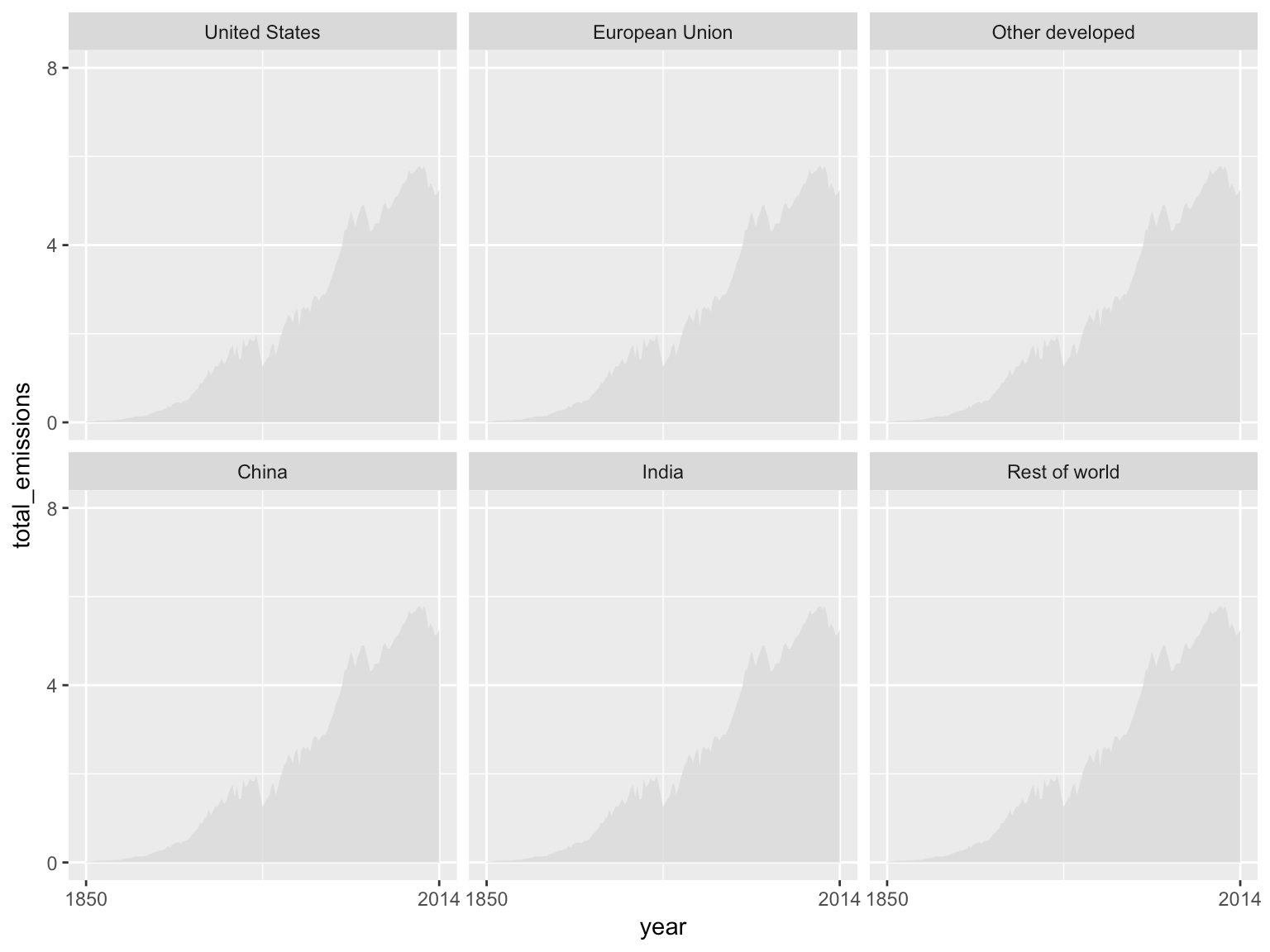Next, let’s remove the background behind the panel headings, the plot and also remove the axis ticks.

`g <- g + theme(strip.background = element_blank(), panel.background = element_blank(), axis.ticks = element_blank())`

Now, we add dotted grid lines for the y-axis:

`g <- g + theme(panel.grid.major.y = element_line(color = "gray85", linetype = "dotted"), panel.grid.minor.y = element_line(color = "gray85", linetype = "dotted"))`

The plot looks like this: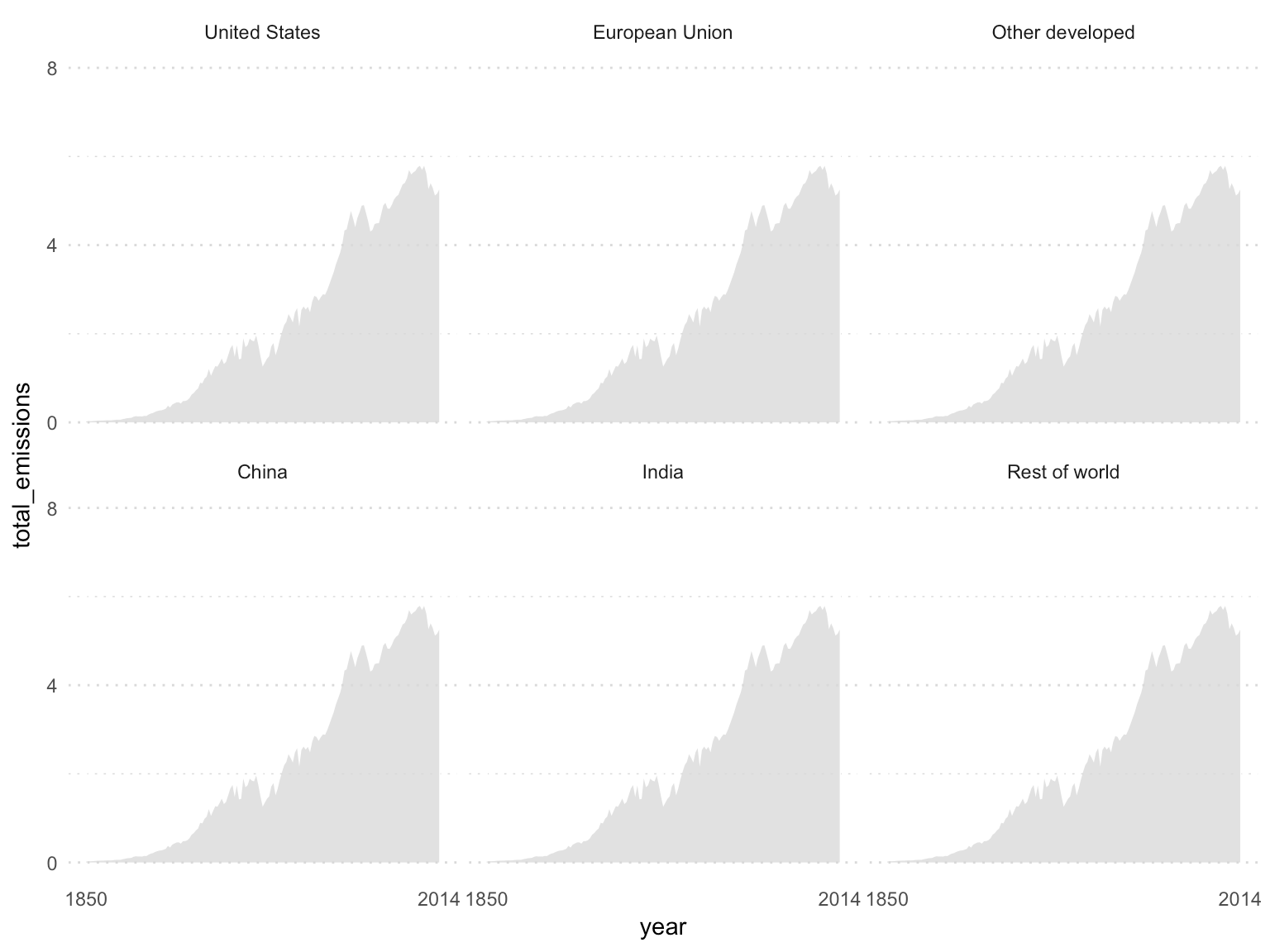Let’s add some space between the panels and improve the readability of the text by increasing the font sizes.

`g <- g + theme(panel.spacing = unit(2, "lines"), strip.text = element_text(size = 12, face = "bold"), axis.title = element_blank(), axis.text = element_text(size = 10))`

Now, let’s plot another layer of data for each of the groups. Since we are plotting this data now, the U.S. data will go in the back, just like the NYT graphic.

`g <- g + geom_area(data = by_chart_groups, aes(x = year, y = total_emissions, fill = economy_type), alpha = 0.9) + scale_fill_manual(values = econ_type_colors, guide = guide_legend(label.position = "right", title = NULL))`

The plot looks like this: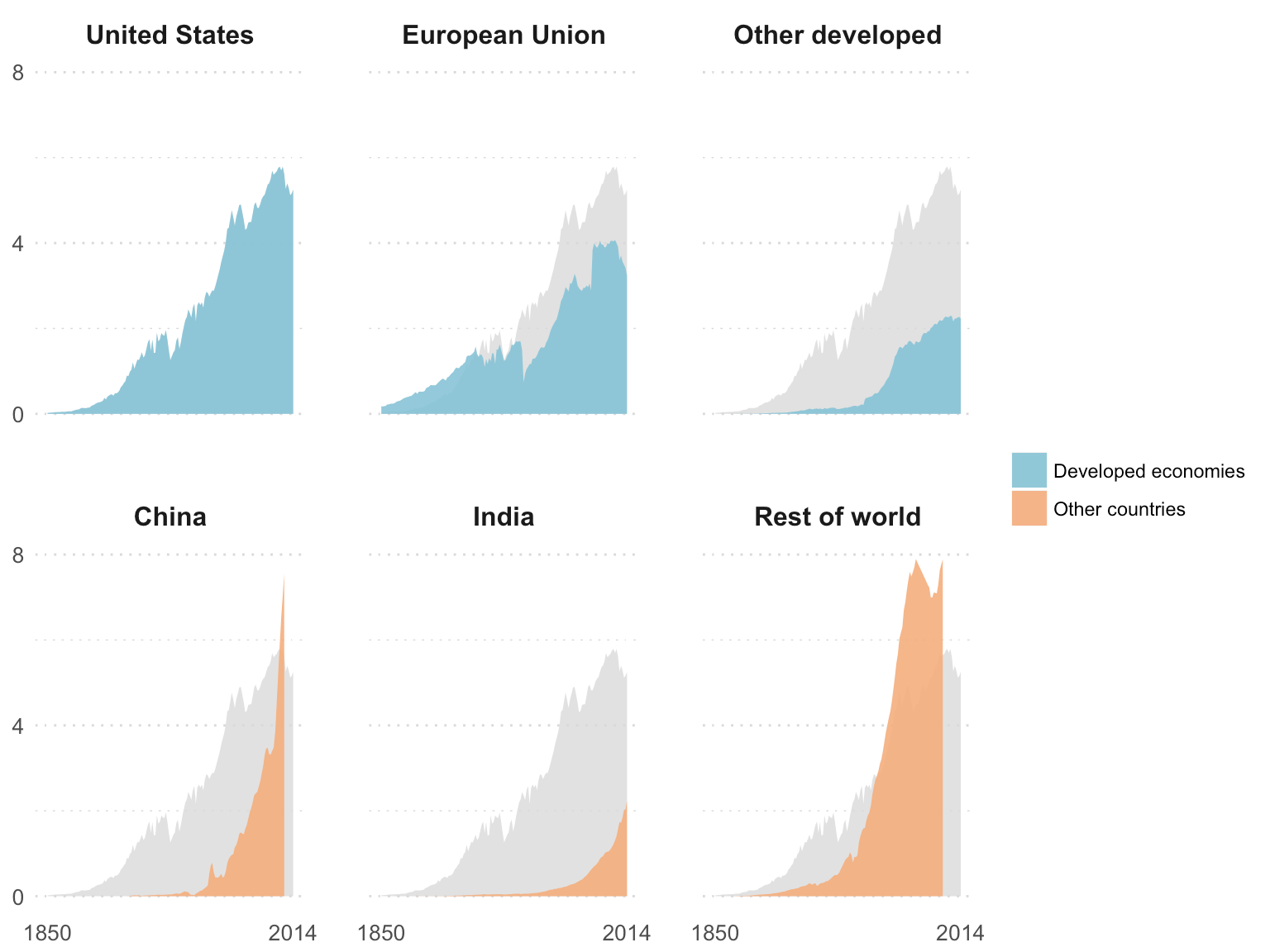Just a few final tweaks to move the legend and add the annotations:

```g <- g + theme(legend.position = "bottom") g <- g + geom_text(data = annotations_txt, aes(x = 1850, y = y, label = txt), size = 3, hjust = "inward", vjust = "inward")```

The final plot looks like this: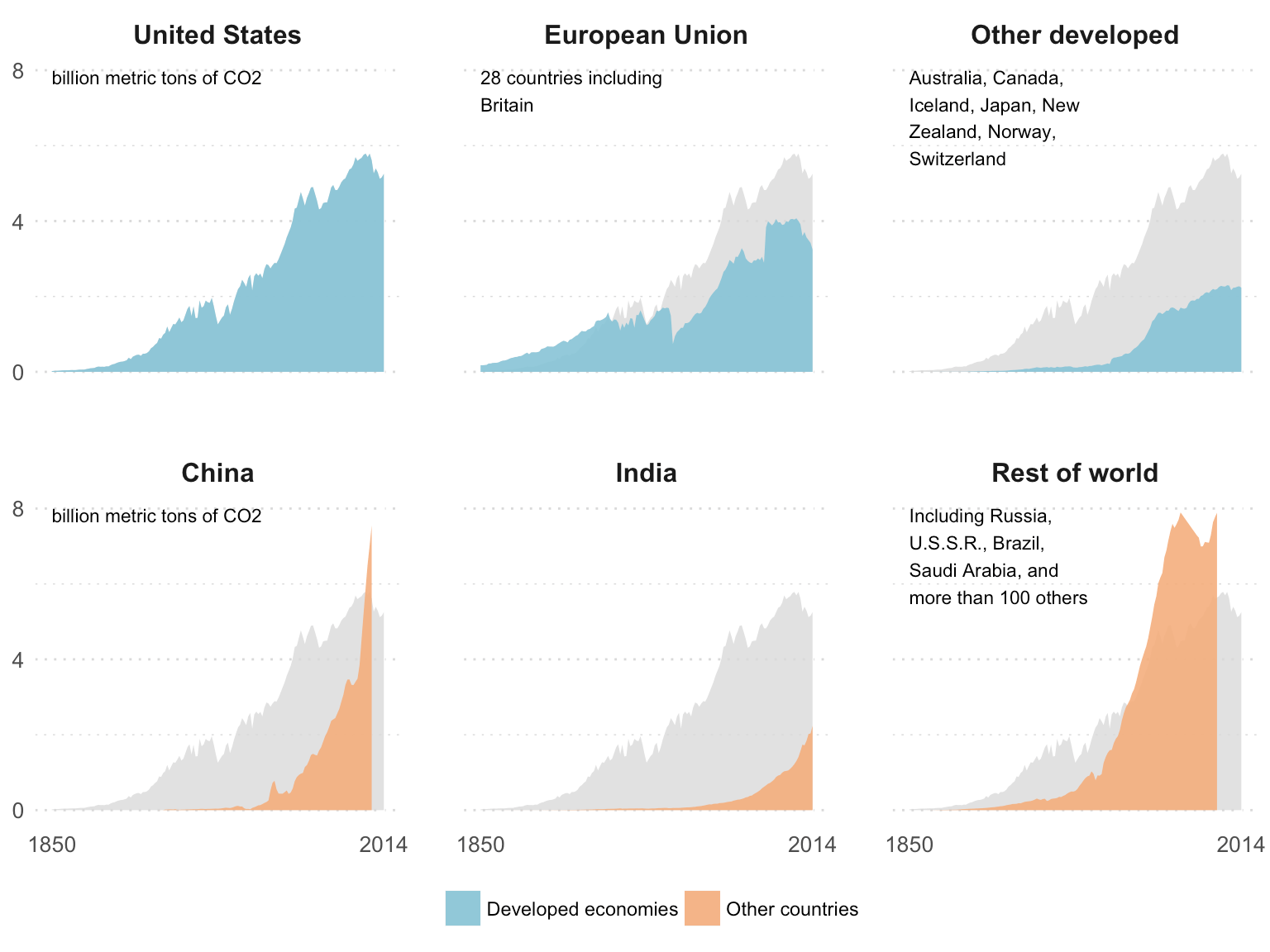Really nice! Don’t you think?## Critique of this data visualization

Although this graph looks pretty, it doesn’t lead to any critical questioning of the issue. Yes, the U.S. emits a lot. Yes, China grew fast and needs a lot of energy. Yet, the graph fails to create an impact. Perhaps, the comparison of the recent years using a line chart or a double-axis chart will show the sudden rise of China. Or, I hate to say it, but an infographic-y think would help in this case. Something like, one person in the US causes more carbon emissions than 10 people in India (just making this up).

just as important it is to “maximize information to pixel ratio”, you should pay special attention to maximize the information to time ratio and get to actionable insights.

## Stacked Area Graph

Unlike the faceted plot, this graph shows all the countries stacked on top of each other.

This graph also shows Russia, so we need to make further manipulations to remove Russia from the Rest of the World category and create its own separate category.

```by_chart_groups_rus <- filter(emissions_by_countries, country != 'Russia') %>% group_by(year, chart_groups, economy_type) %>% summarize(total_emissions = sum(co2_total_emissions*10^3, na.rm = TRUE)) %>% ungroup() %>% bind_rows(., filter(emissions_by_countries, country == 'Russia') %>% group_by(year, economy_type) %>% summarize(total_emissions = sum(co2_total_emissions*10^3, na.rm = TRUE)) %>% ungroup() %>% mutate(chart_groups = 'Russia')) %>% mutate(chart_groups = factor(chart_groups, levels = c('Rest of world', 'India', 'China', 'Russia', 'Other developed', 'European Union', 'United States'))) %>% arrange(year, chart_groups)```

This data frame looks like this:

`glimpse(by_chart_groups_rus)`
``````## Observations: 1,287
## Variables: 4
## \$ year             1751, 1752, 1753, 1754, 1755, 1756, 1757, 1758...
## \$ chart_groups     European Union, European Union, European Unio...
## \$ economy_type     "Developed economies", "Developed economies", ...
## \$ total_emissions  9358184, 9361851, 9361851, 9365518, 9369185, 1...``````

Let’s create this graph now:

```g <- ggplot(by_chart_groups_rus, aes(x = year, y = total_emissions, fill = chart_groups)) + geom_area(color = "white", size = 0.3) g <- g + scale_fill_manual(values = c(rep("#F4AE7B", 4), rep("#86C3D6", 3)), guide = FALSE) g <- g + scale_x_continuous(limits = c(1850, 2014), breaks = seq(from = 1850, to = 2014, by = 50), expand = c(0, 0)) + scale_y_continuous(position = "right", expand = c(0, 0), labels = formatter_billions, breaks = seq(from = 0, to = 35, by = 5)*10^9)```

Similar to the graph above, we create an area graph, but instead of creating facets, we use the categories to fill with a color. We also add some separation between the plots using the white color. Also, notice the `position = "right"` argument in the `scale_y_continuous` function; this will move the y-axis to the right.

This graph looks like this: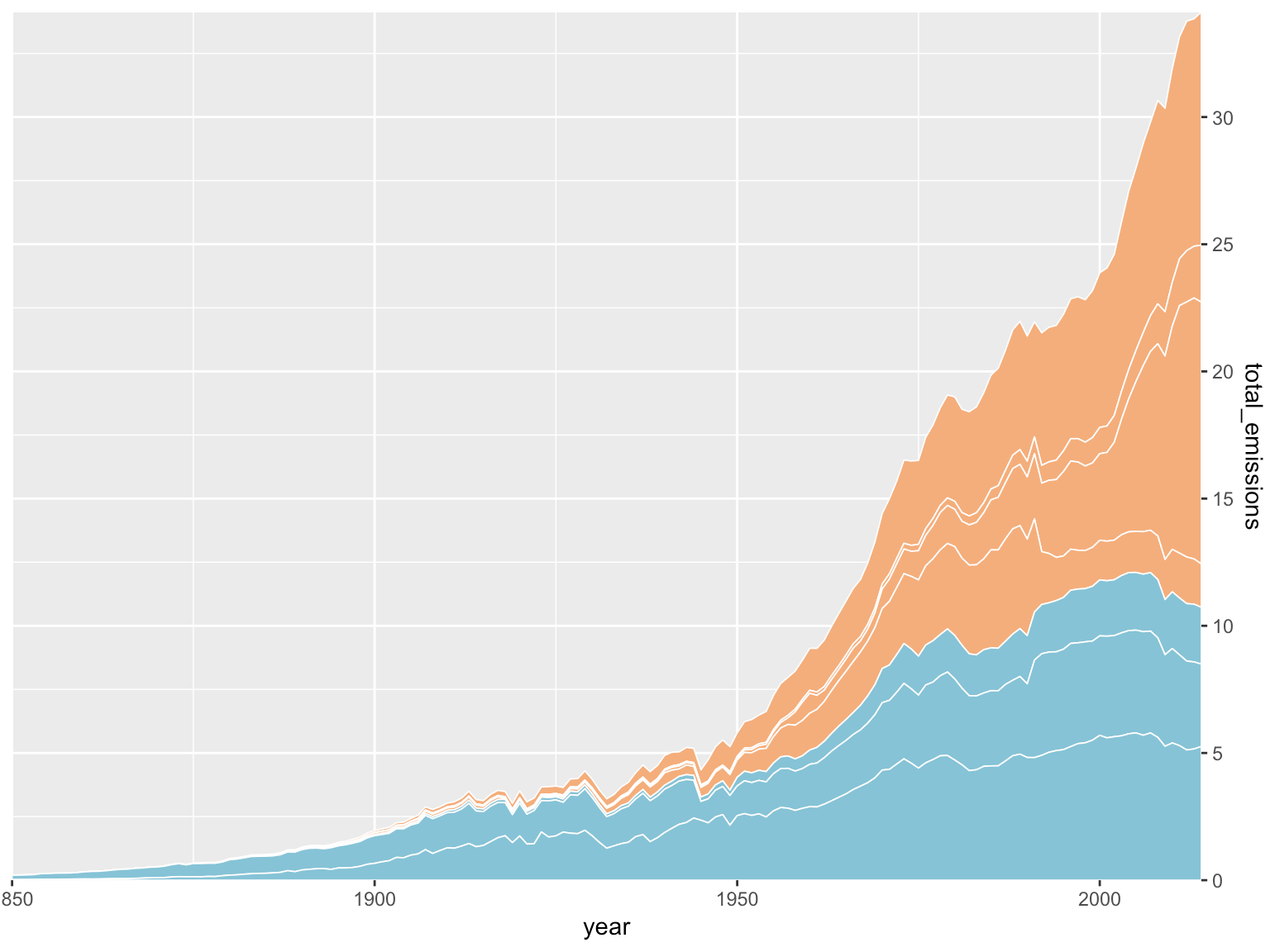Now, let’s change the color of the axis labels and add some more space around the plot:

```g <- g + theme(panel.background = element_blank(), axis.ticks.length = unit(5, "points"), axis.text = element_text(color = "grey70", size = 10), axis.ticks = element_line(color = "grey70")) g <- g + theme(axis.title = element_blank(), plot.margin = unit(c(0, 25, 15, 15), "points"))```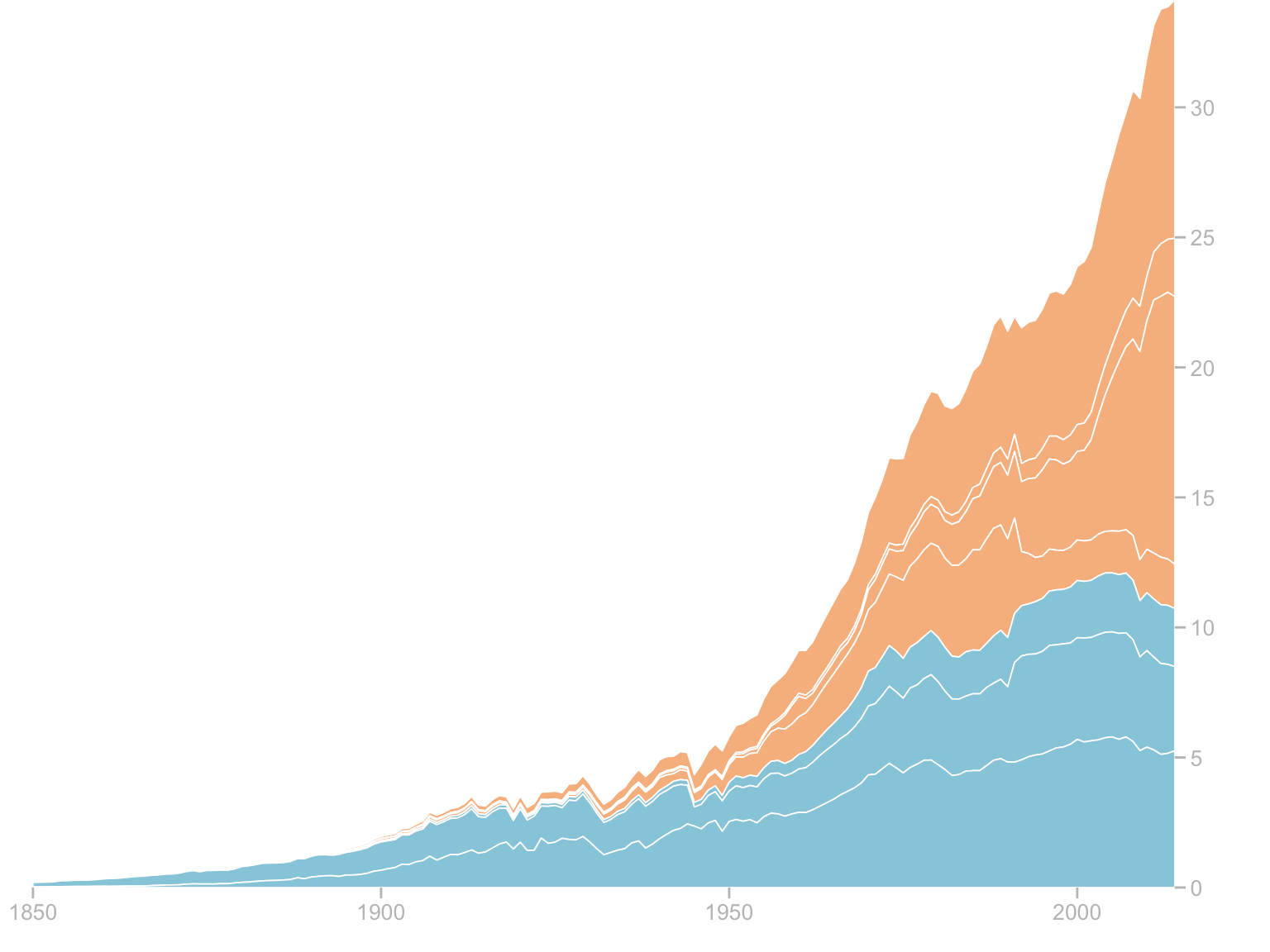Last step is adding the country names to the areas. I created a separate data frame with the country names and positions. For the most part, I used the cumulative total of a country to decide the location, but I had to manually add a few locations:

```ann_text <- filter(by_chart_groups_rus, year == 2014) %>% arrange(desc(chart_groups)) %>% mutate(cumtot = cumsum(total_emissions)) %>% select(chart_groups, y = cumtot) %>% mutate(x = 2000) %>% mutate(x = ifelse(chart_groups == 'India', 2010, x), y = ifelse(chart_groups == 'India', 23.5*10^9, ifelse(chart_groups == 'China', 16*10^9, ifelse(chart_groups == 'Rest of world', 30*10^9, y)))) g <- g + geom_text(data = ann_text, aes(x = x, y = y, label = chart_groups), vjust = 1) g <- g + annotate(geom = "text", x = 1925, y = 25*10^9, label = "CO2 emitted worldwide", fontface = 2, hjust = 0) g <- g + annotate(geom = "text", x = 1925, y = 24*10^9, label = "Between 1850-2014", hjust = 0, vjust = 0)```

The final plot looks like this: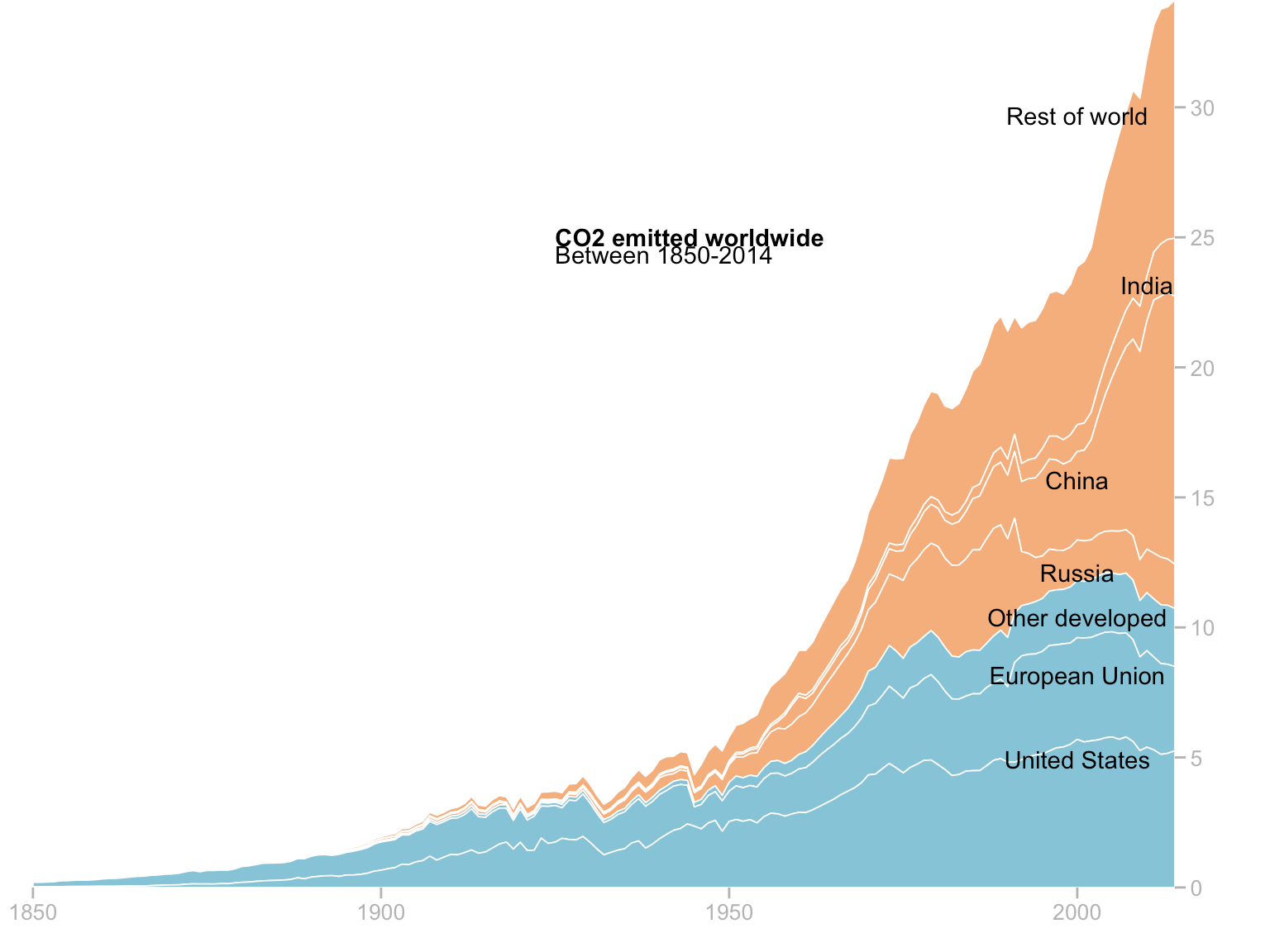## Critique of this data visualization

What’s worse than an area chart: a stacked area chart. Although stacked area charts (or bar charts) let us see the cumulative totals and proportions of each category, the readers have a tough time comparing the proportions and telling the differences. Faceted or paneled line charts by country could be a good choice. We can also consider a slopegraph.## Get the top 10 by per capita

Next graph in the NYT’s chart is the bar graph of per capita emissions for a select few countries. I selected the top 10 emitters:

`top_per_capity_country <- filter(emissions_by_countries, year == 2014) %>% top_n(n = 10, wt = co2_per_capita_emission_rate)`

Then, I plotted them as a bar graph

```g <- ggplot(top_per_capity_country, aes(x = reorder(country, co2_per_capita_emission_rate), y = co2_per_capita_emission_rate, fill = economy_type)) + geom_bar(stat = "identity") + coord_flip() g <- g + theme(panel.background = element_blank(), axis.title = element_blank(), axis.ticks.y = element_blank(), axis.text.y = element_text(hjust = 0, color = "black", size = 12), axis.ticks.x = element_blank(), axis.text.x = element_blank()) g <- g + scale_fill_manual(values = econ_type_colors, guide = FALSE) g <- g + ggtitle("Per person carbon emissions in 2014") + theme(title = element_text(face = "bold", size = 10, hjust = 0)) g <- g + geom_text(aes(y = co2_per_capita_emission_rate, x = country, label = round(co2_per_capita_emission_rate, digits = 1)), nudge_y = -1)```

This looks very different from NYT’s chart. These are not the top 10 in its chart.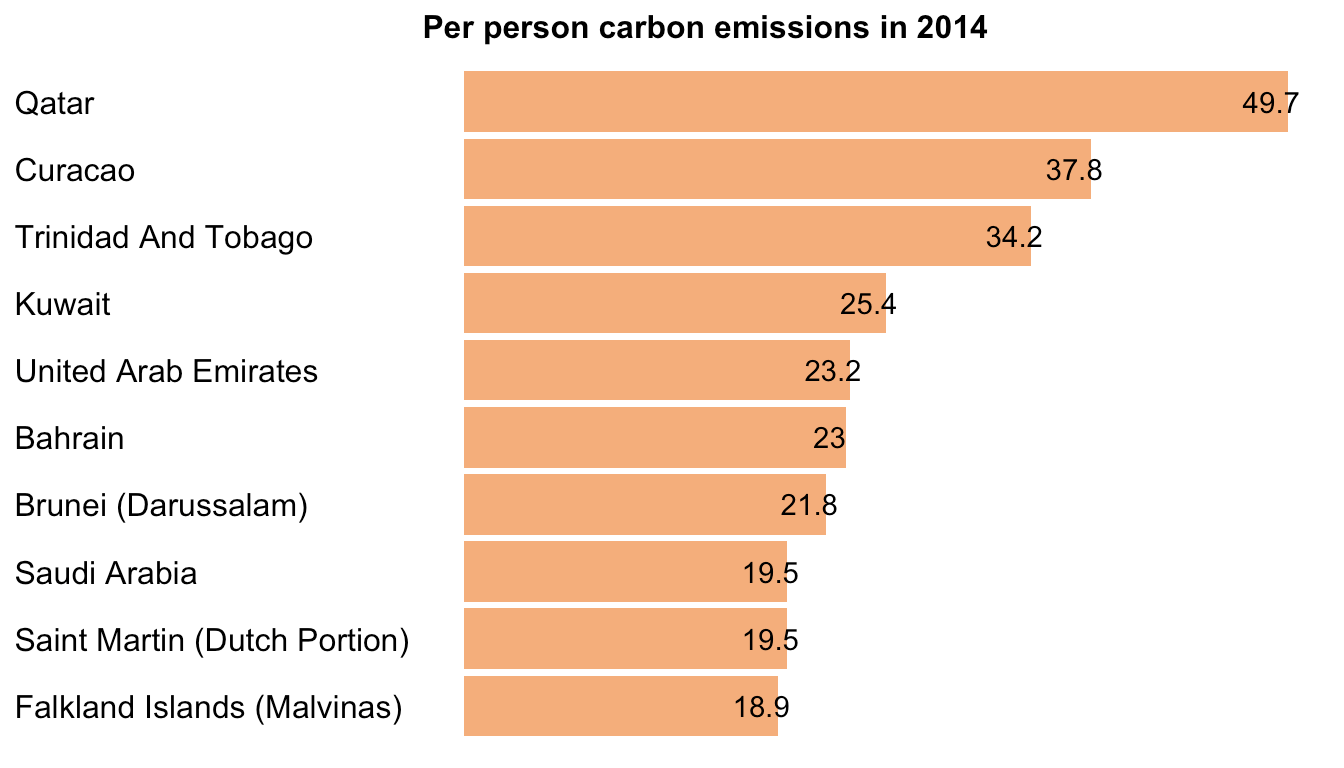## Get the top as shown in NYT

I guess the NYT wanted to show these specific countries, so I selected them manually here:

```nyt_top_10 <- c('United States Of America', 'Canada', 'Russia', 'Japan', 'Germany', 'China', 'United Kingdom', 'France', 'Mexico', 'Brazil', 'India') top_per_capity_country <- filter(emissions_by_countries, year == 2014 &amp; country %in% nyt_top_10)```

And plotted it again:

```g <- ggplot(top_per_capity_country, aes(x = reorder(country, co2_per_capita_emission_rate), y = co2_per_capita_emission_rate, fill = economy_type)) + geom_bar(stat = "identity") + coord_flip() g <- g + theme(panel.background = element_blank(), axis.title = element_blank(), axis.ticks.y = element_blank(), axis.text.y = element_text(hjust = 0, color = "black", size = 12), axis.ticks.x = element_blank(), axis.text.x = element_blank()) g <- g + scale_fill_manual(values = econ_type_colors, guide = FALSE) g <- g + ggtitle("Per person carbon emissions in 2014") + theme(title = element_text(face = "bold", size = 10, hjust = 0)) g <- g + geom_text(aes(y = co2_per_capita_emission_rate, x = country, label = round(co2_per_capita_emission_rate, digits = 1)), nudge_y = -0.5)```

This is how the NYT bar chart looks like: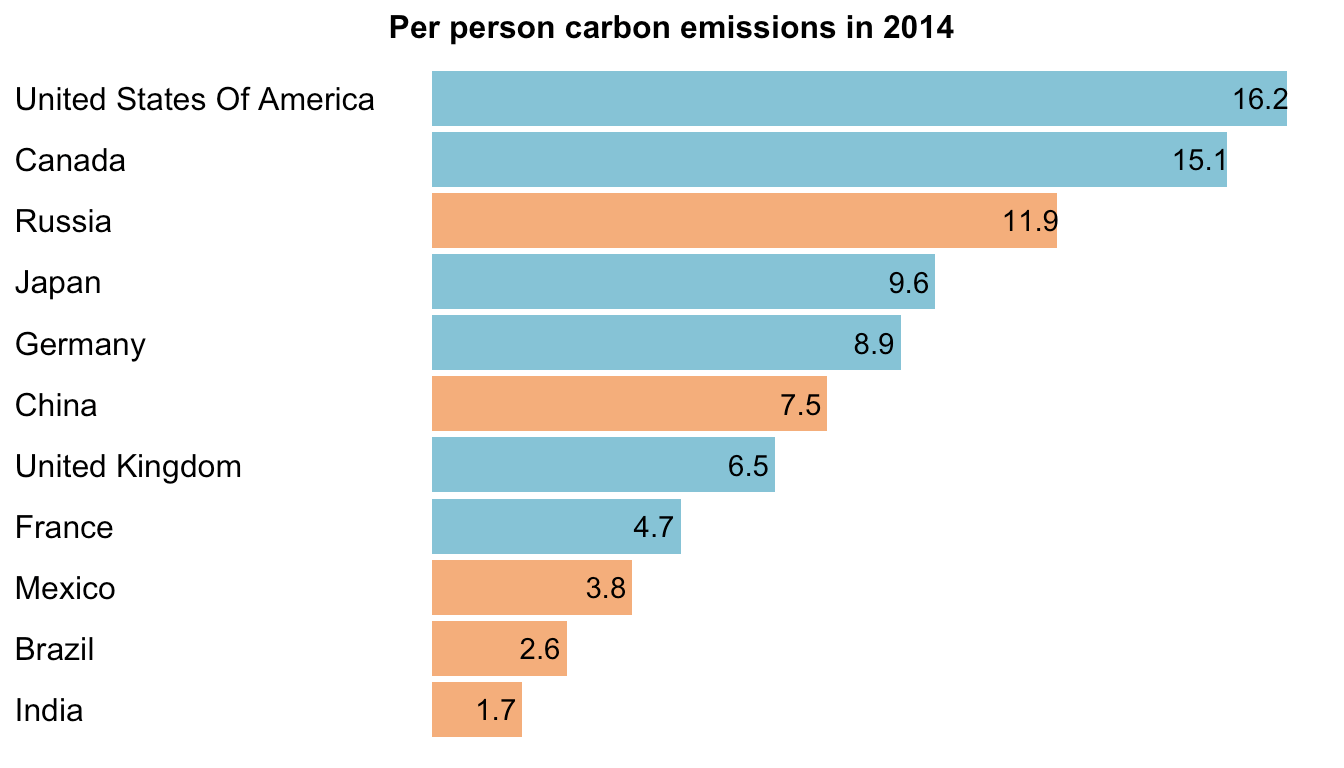## Add axis back and grid lines

I would argue that having an axis along with almost invisible grid lines make this chart look better. Also, I would argue that listing the data point and plotting the chart is redundant. But, in this case, we will just go with it.

```g <- g + theme(axis.ticks.x = element_line(color = "gray80"), axis.text.x = element_text(color = "black")) g <- g + geom_hline(yintercept = seq(from = 5, to = 15, by = 5), color = "gray85")```

This is how the modified NYT bar chart looks like: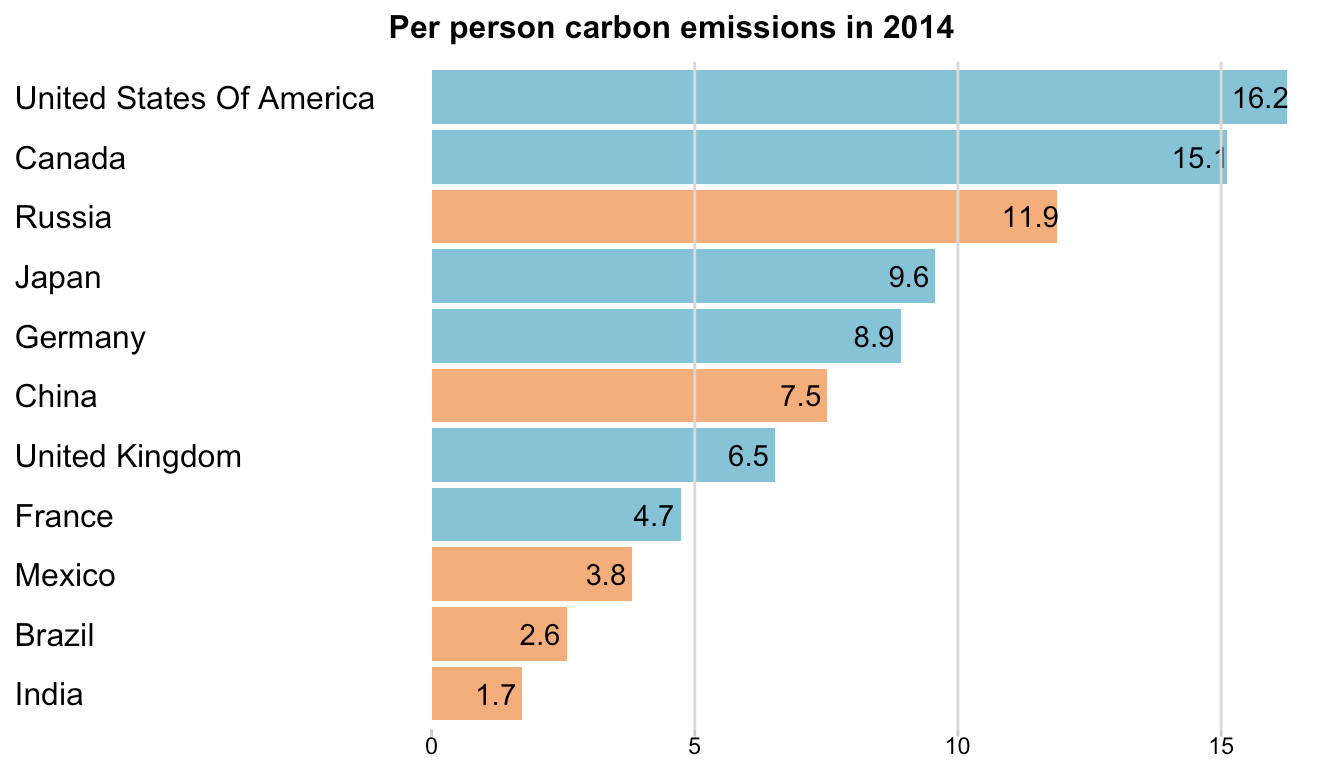I think it adds more clarity to the data visualization. What do you think?

## Seeing data differently

What if we were to see all the countries as line charts instead of area charts for a select few, do you think it will tell a different story? I wanted to find out.

First, I got only 2014 data for each country:

`last_year_emission <- filter(emissions_by_countries, year == 2014) %>% select(year, country, co2_total_emissions) %>% mutate(totem = co2_total_emissions*10^3)`

Then I plotted this data along with the labels:

```g <- ggplot(emissions_by_countries, aes(x = year, y = co2_total_emissions*10^3, group = country, color = economy_type, label = country)) + geom_line(size = 1) + geom_text(data = last_year_emission, aes(x = year, y = totem, label = country), nudge_x = 2, hjust = 0, check_overlap = TRUE, inherit.aes = FALSE) + scale_x_continuous(expand = c(0.2, 0)) g <- g + scale_color_manual(values = econ_type_colors, guide = FALSE) g <- g + scale_y_continuous(expand = c(0.05, 0), labels = formatter_billions) g <- g + theme(panel.background = element_blank(), axis.text = element_text(color = "grey70", size = 10), axis.ticks = element_blank(), axis.title = element_blank()) g <- g + theme(plot.margin = unit(c(2, 1, 1, 1), "lines"))```

Here’s how all the lines shape up: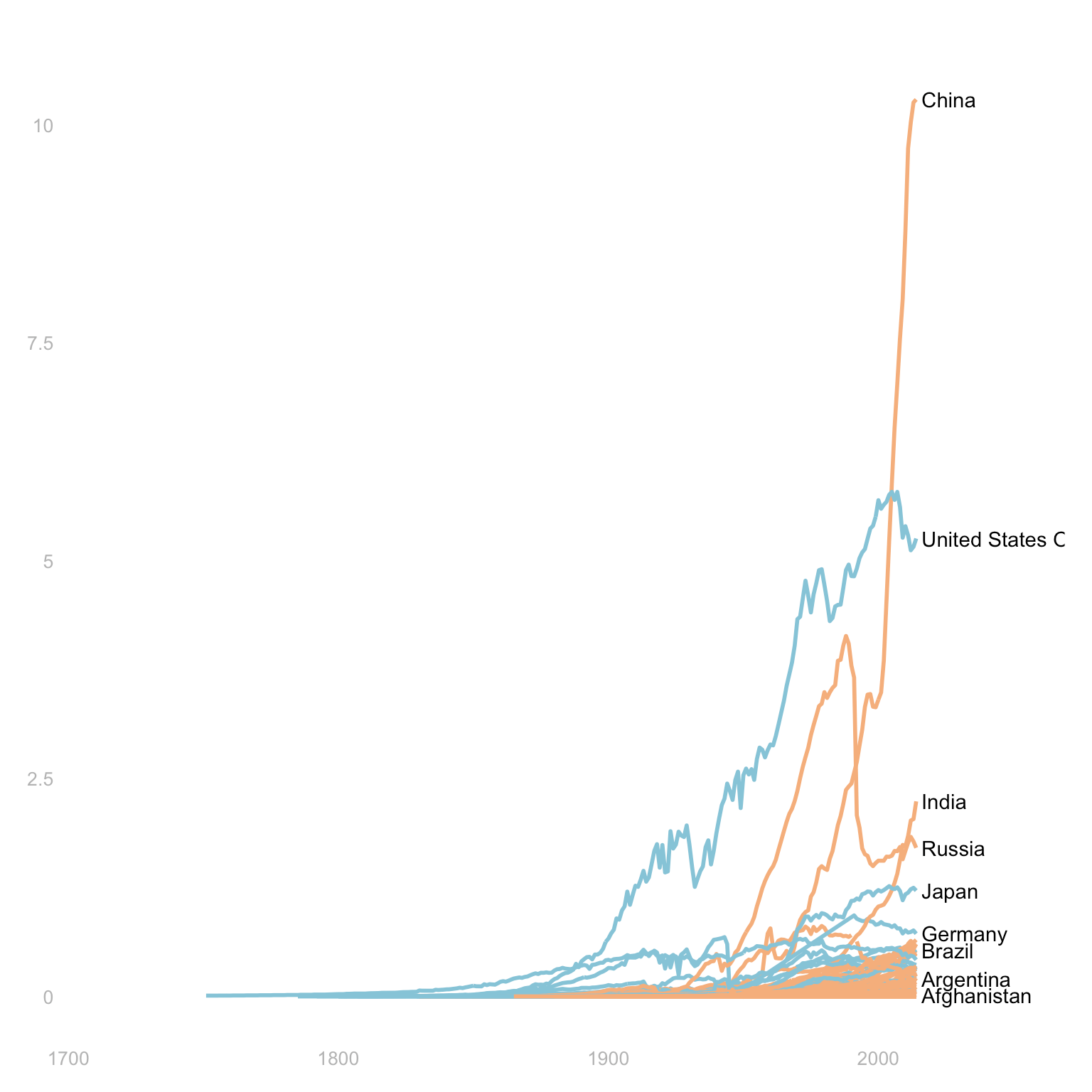Although you can’t see all the individual country lines at the bottom, you can see the clear difference in the biggest and smaller emitters.

## Focusing on the past 30 years

Do the trends differ if we look at only the past 30 years?

```g <- ggplot(filter(emissions_by_countries, year > 1984), aes(x = year, y = co2_total_emissions*10^3, group = country, color = economy_type, label = country)) + geom_line(size = 1) + geom_text(data = last_year_emission, aes(x = year, y = totem, label = country), nudge_x = 2, hjust = 0, check_overlap = TRUE, inherit.aes = FALSE) + scale_x_continuous(expand = c(0.2, 0)) g <- g + scale_color_manual(values = econ_type_colors, guide = FALSE) g <- g + scale_y_continuous(expand = c(0.05, 0), labels = formatter_billions) g <- g + theme(panel.background = element_blank(), axis.text = element_text(color = "grey70", size = 10), axis.ticks = element_blank(), axis.title = element_blank()) g <- g + theme(plot.margin = unit(c(2, 1, 1, 1), "lines"))```

The past 30 years show the sudden increase in China’s emissions compared to the emissions of the rest of the countries: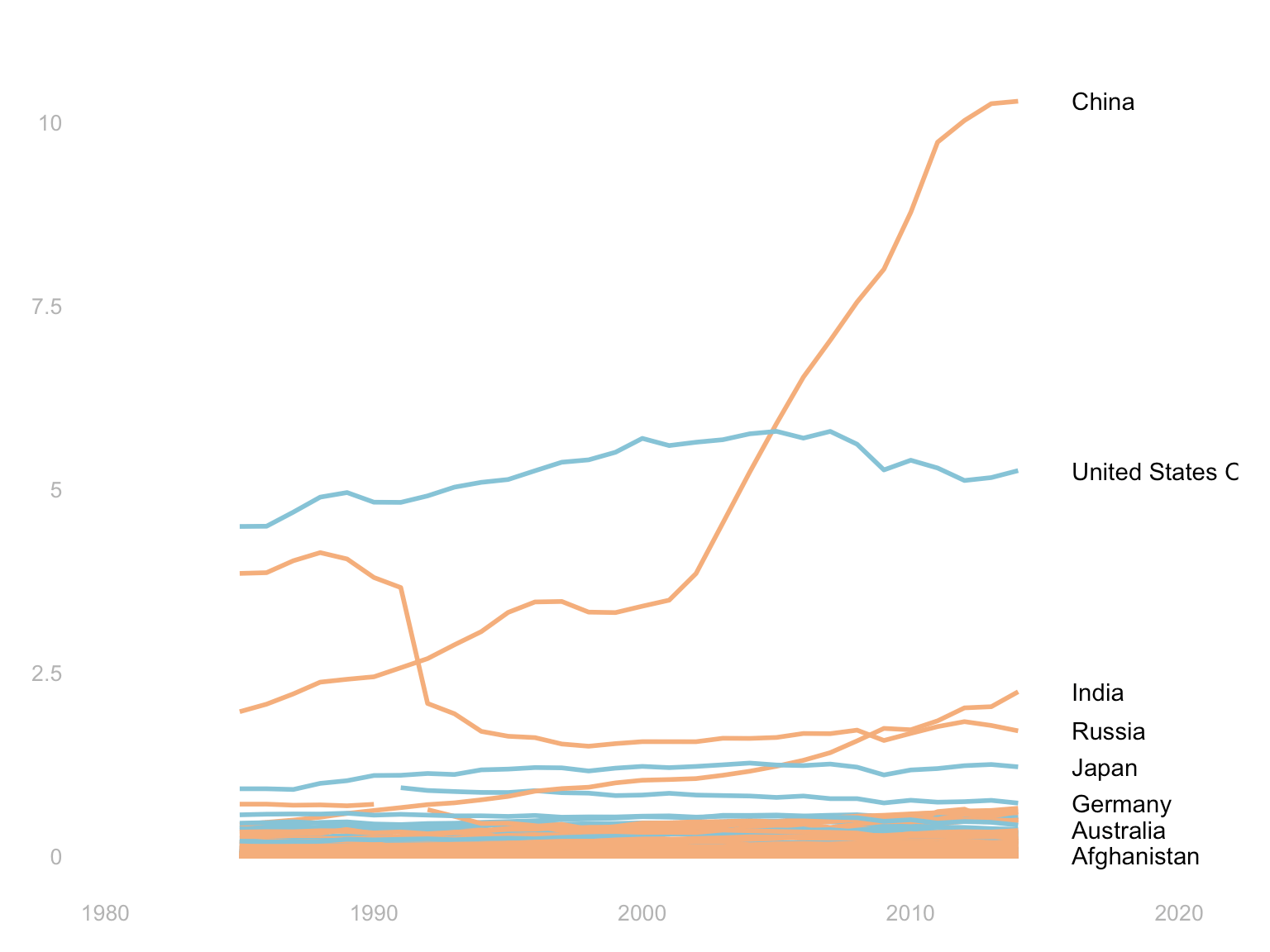# Washington Post Graphics

The Washington Post used similar data and created its own graphs:

The WaPo graphic makes a point by separating the countries by their participation in the Paris agreement. The U.S. doesn’t have a good company in Nicaragua and Syria.

```last_year_emission <- filter(emissions_by_countries, year == 2014) %>% mutate(totem = co2_total_emissions*10^3, paris_agreement = ifelse(country %in% c('United States Of America', 'Nicaragua', 'Syrian Arab Republic'), 'NOT PART OF AGREEMENT', 'PART OF AGREEMENT'))```

At first, I tried the packed bubble graph using the `D3` import in the library `bubbles`

```library(bubbles) with(last_year_emission, bubbles(value = totem, label = country, color = econ_type_colors))```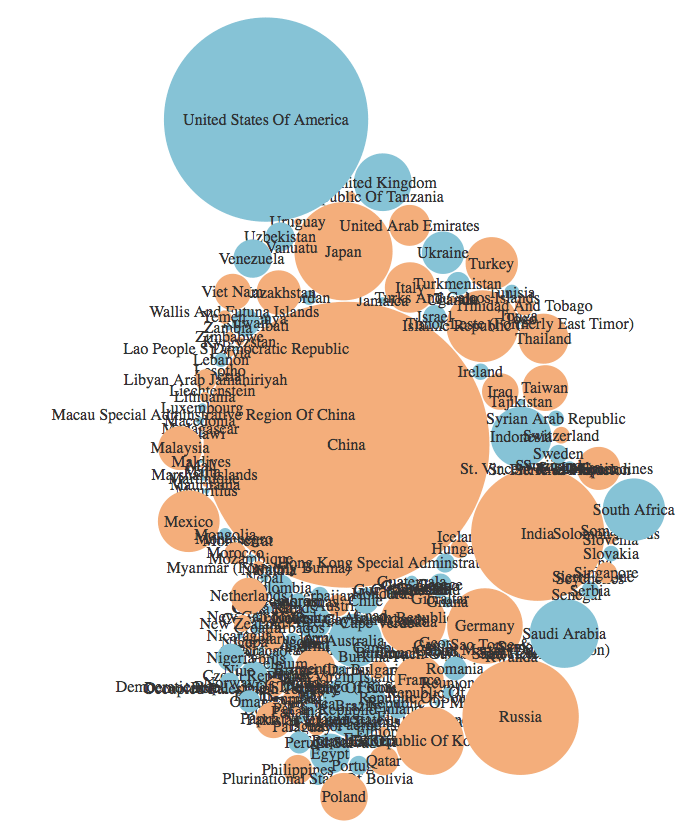That’s doesn’t look too good. Let’s try `ggplot`. The WaPo graphic has the filled circles of countries neatly laid out. And, if you notice, there are no axes. We have two options: either hard-code every location of a country, or, randomly divide the countries.

I don’t want to hard-code every single location of the country, so I tried to create some groupings of five countries each. I gave group numbers to all the countries ordered descending by the total emissions. Then, I created 20 bins using quartiles to put each country in a category.

```last_year_emission <- last_year_emission %>% arrange(desc(paris_agreement), desc(totem)) %>% mutate(group_num = rep(1:5, 44), y = cut(totem, breaks = quantile(totem, probs = seq(0, 1, 0.05)))) %>% filter(!(is.na(y)))```
```g <- ggplot(data = last_year_emission, aes(x = group_num, y = y, size = totem, fill = economy_type, label = country)) + geom_jitter(shape = 21, alpha = 0.8) + scale_size(range = c(2, 30)) + geom_text(size = 3, check_overlap = TRUE) + scale_fill_manual(values = econ_type_colors) + facet_wrap(~paris_agreement) g <- g + theme(panel.background = element_blank(), axis.text = element_blank(), axis.title = element_blank(), axis.ticks = element_blank()) + guides(size = FALSE, fill = FALSE) g <- g + scale_y_discrete(expand = c(0.1, 0)) + theme(strip.background = element_blank()) + ggtitle("Total CO2 Emissions 2014")```

Here how it looks: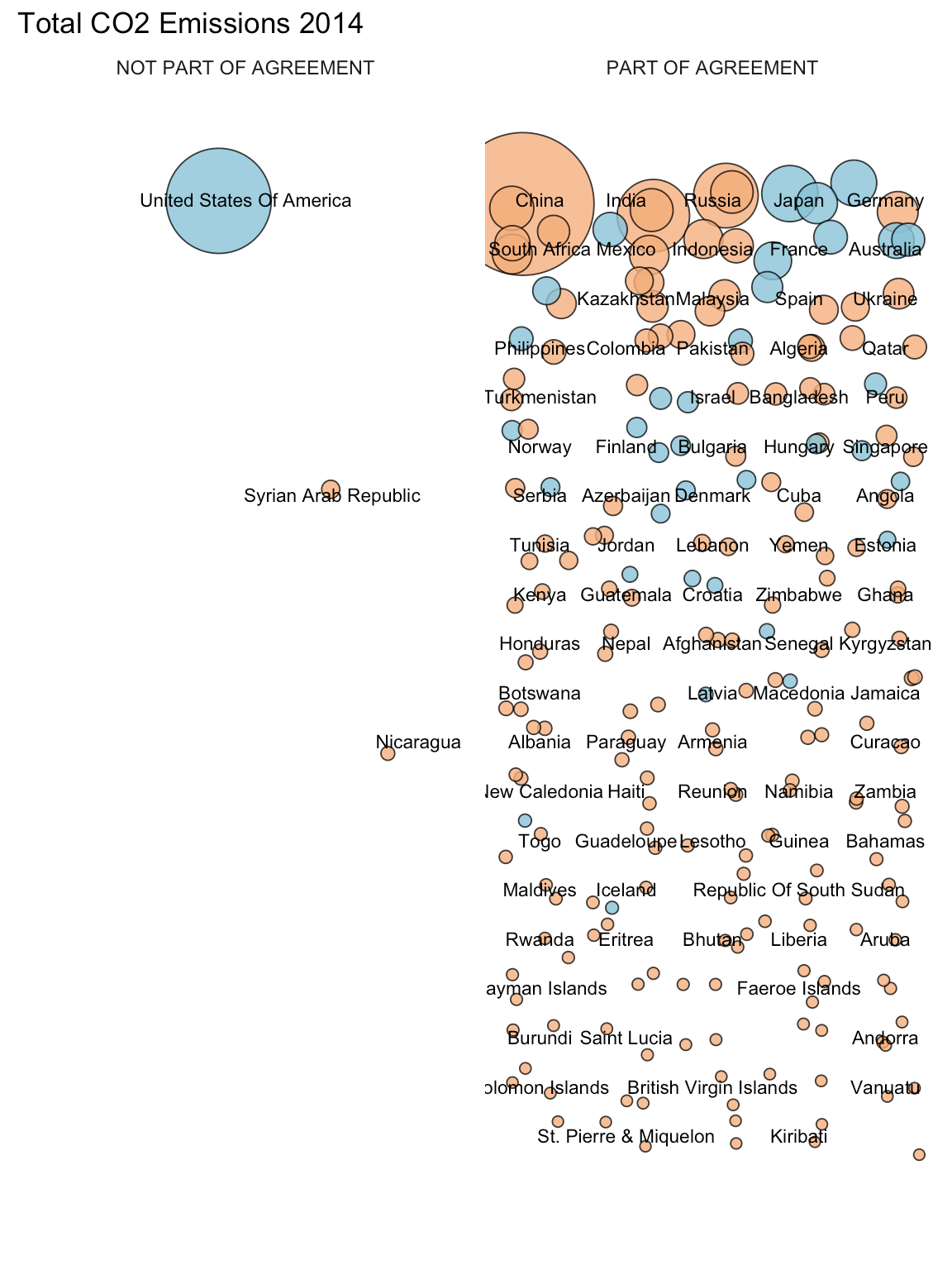Arrggh. That’s ugly. Yoda be like:The binning didn’t work out.

Let’s try log transformation of the total emissions

```g <- ggplot(data = last_year_emission, aes(x = group_num, y = totem, size = totem, fill = economy_type, label = country)) + geom_point(shape = 21, alpha = 0.8) + scale_size(range = c(2, 30)) + geom_text(size = 3, check_overlap = TRUE) + scale_fill_manual(values = econ_type_colors) + facet_wrap(~paris_agreement) g <- g + theme(panel.background = element_blank(), axis.text = element_blank(), axis.title = element_blank(), axis.ticks = element_blank()) + guides(size = FALSE, fill = FALSE) g <- g + scale_x_continuous(expand = c(0.5, 0)) + scale_y_log10(expand = c(0.01, 0.5)) + theme(strip.background = element_blank()) + ggtitle("Total CO2 Emissions 2014")```

Log transformation looks like this: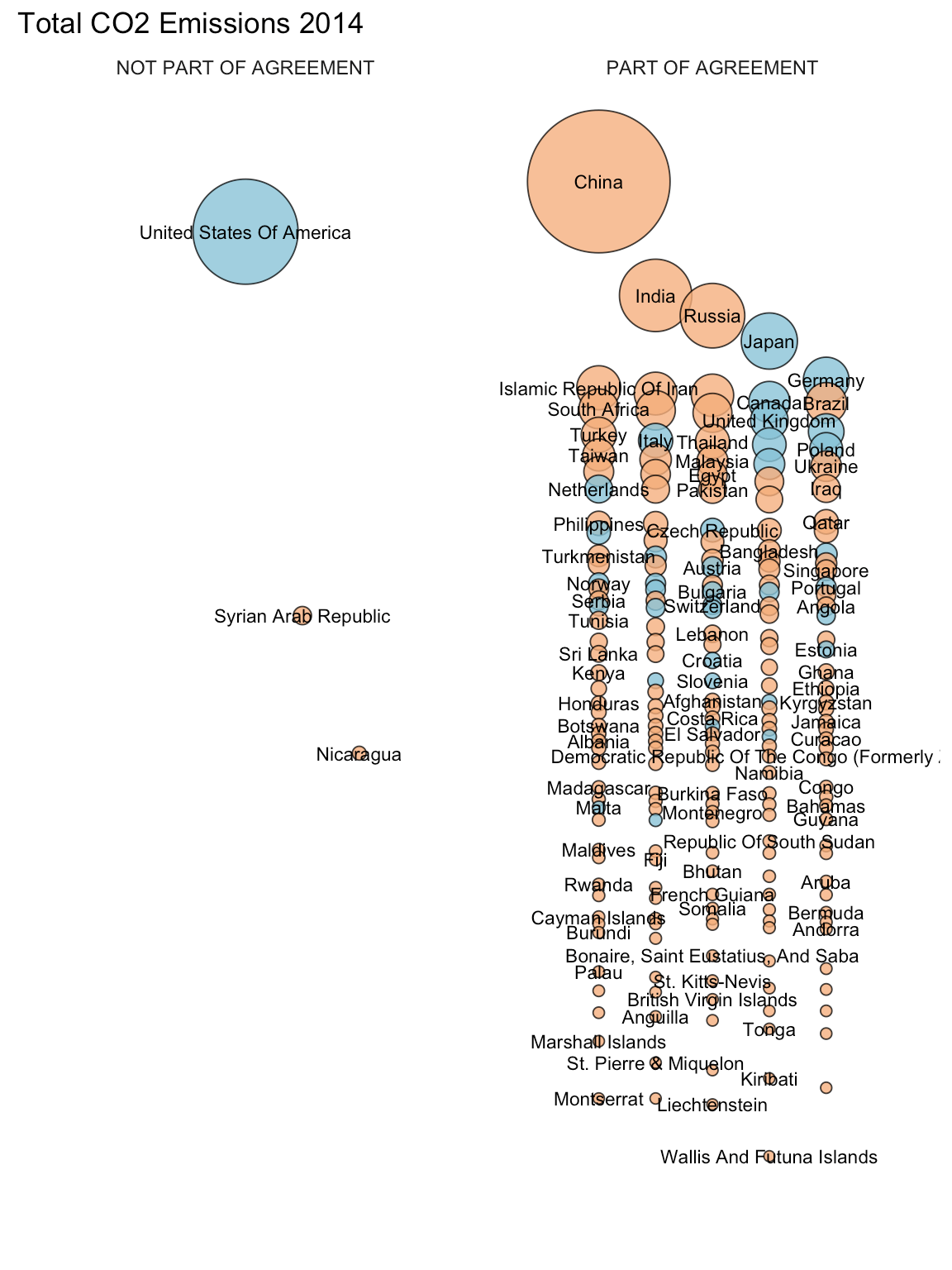Better, but still ugly.

Let’s try a clustering way. Let’s create a few clusters first:

```emission_clusters <- kmeans(select(last_year_emission, -country, -year, -(EU:y)), 10) last_year_emission <- mutate(last_year_emission, emission_cluster = emission_clusters\$cluster)```

Then use these cluster values to distribute the countries on the x-axis.

```g <- ggplot(data = last_year_emission, aes(x = emission_cluster, y = totem, size = totem, fill = economy_type, label = country)) + geom_jitter(shape = 21, alpha = 0.8) + scale_size(range = c(2, 30)) + geom_text(size = 3, check_overlap = TRUE) + facet_wrap(~paris_agreement) + scale_fill_manual(values = econ_type_colors) g <- g + theme(panel.background = element_blank(), axis.text = element_blank(), axis.title = element_blank(), axis.ticks = element_blank()) + guides(size = FALSE, fill = FALSE) g <- g + scale_x_continuous(expand = c(0.5, 0)) + scale_y_log10(expand = c(0.01, 0.5)) + theme(strip.background = element_blank()) + ggtitle("Total CO2 Emissions 2014")```

It looks like this: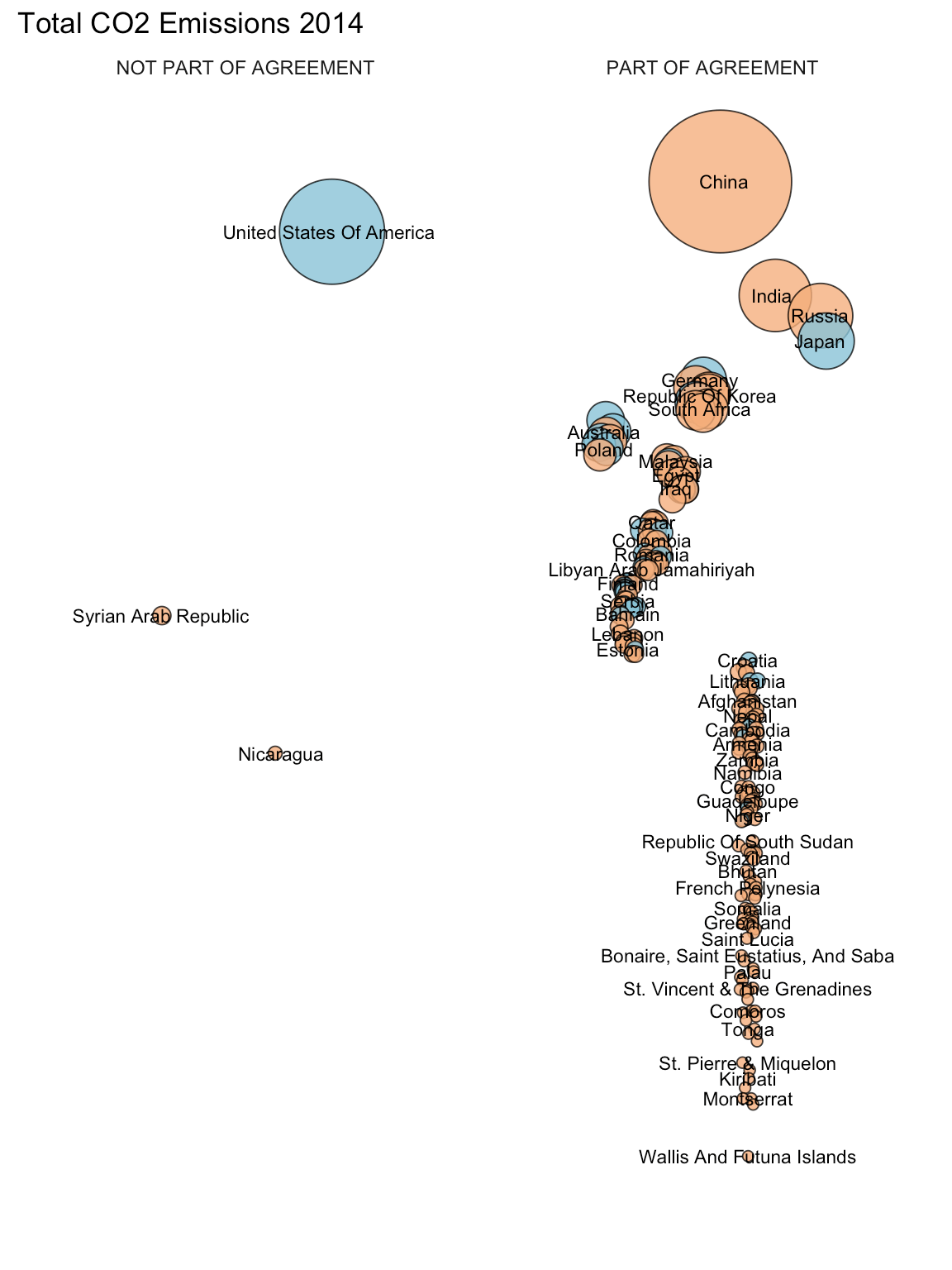Still not good. Mr. Bean doesn’t approve:Then I found a library called `packcircles`, which creates Tableau-like packed circles/bubbles.

`library(packcircles)`

Need to get the colors column in the last year emission data frame:

`last_year_emission <- left_join(last_year_emission, data.frame(economy_type = names(econ_type_colors), color = econ_type_colors, row.names = NULL, stringsAsFactors = FALSE), by = "economy_type") %>% arrange(desc(paris_agreement), desc(totem))`

Create the circle layout:

`last_year_emission_cir_layout <- circleProgressiveLayout(last_year_emission, sizecol = 'totem')`

Which returns the x,y coordinates:

`glimpse(last_year_emission_cir_layout)`
``````## Observations: 217
## Variables: 3
## \$ x       -57236.545, 26692.650, 8478.961, 7154.090, 41817.531, 6...
## \$ y       0.000, 0.000, -46555.283, 42031.453, 39000.068, 18710.6...
## \$ radius  57236.545, 26692.650, 23298.678, 19658.169, 15137.570, ...``````

Then, compute the vertices of these circles:

`last_year_emission_cir_layout_vertices <- circleLayoutVertices(last_year_emission_cir_layout, npoints = 50)`
`glimpse(last_year_emission_cir_layout_vertices)`
``````## Observations: 11,067
## Variables: 3
## \$ x   0.0000, -451.3273, -1798.1913, -4019.3513, -7079.7783, -109...
## \$ y   0.000, 7173.641, 14234.150, 21070.177, 27573.916, 33642.797...
## \$ id  1, 1, 1, 1, 1, 1, 1, 1, 1, 1, 1, 1, 1, 1, 1, 1, 1, 1, 1, 1,...``````

Then, the fun part, plot it:

```g <- ggplot(data = last_year_emission_cir_layout_vertices) + geom_polygon(aes(x, y, group = id, fill = factor(id)), color = "black", show.legend = FALSE, alpha = 0.8) g <- g + scale_fill_manual(values = last_year_emission\$color) + coord_equal() + geom_text(data = last_year_emission_cir_layout, aes(x, y), label = last_year_emission\$country, check_overlap = TRUE) g <- g + theme(axis.title = element_blank(), axis.ticks = element_blank(), axis.text = element_blank(), panel.background = element_blank()) + scale_x_continuous(expand = c(0.1, 0))```

Which looks like this: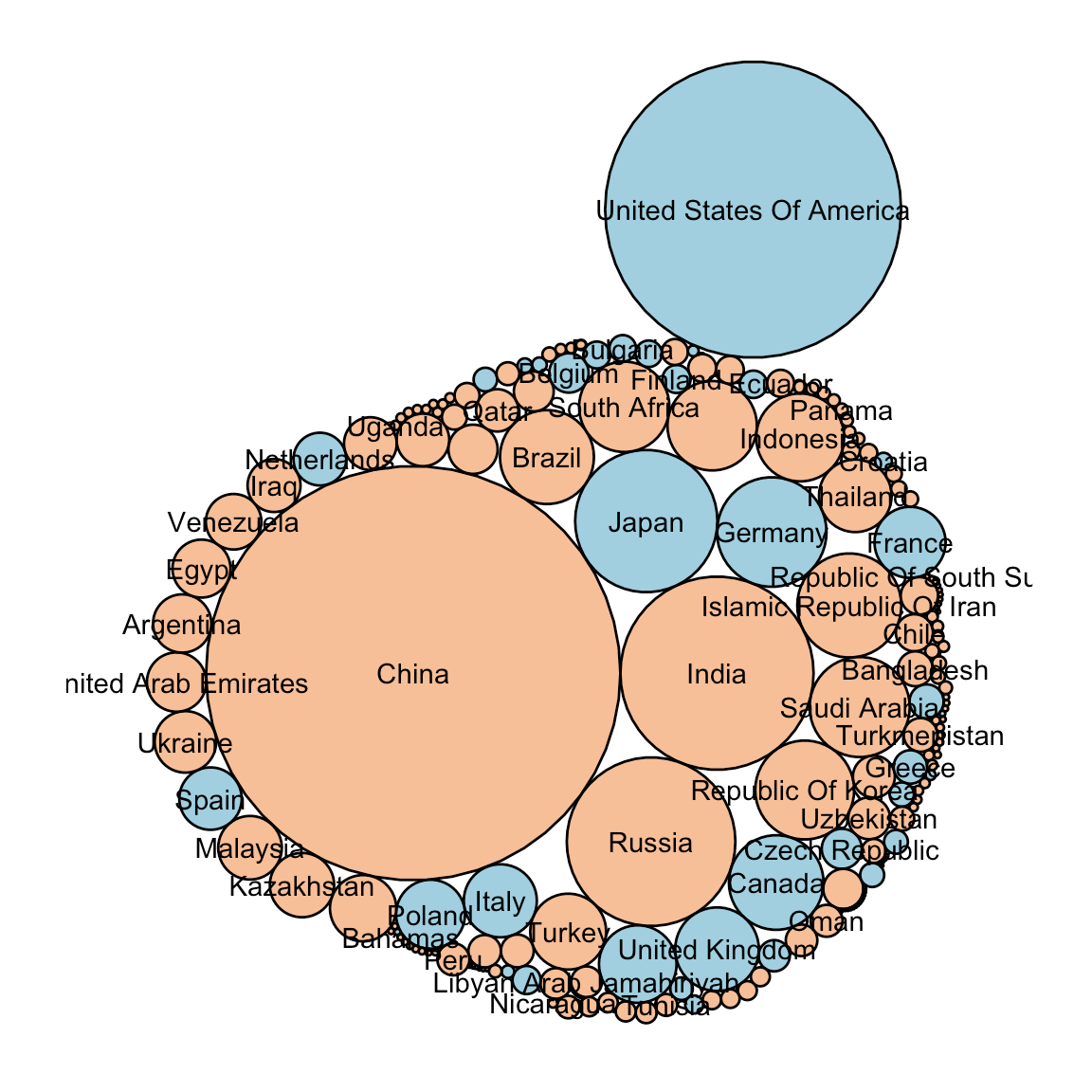Much better, no?

I wish there was some great learning here rather than knowing the already-known big emitters. Can you think of any other ways to explore this data set and find something that we already didn’t know? Let me know in the comments.

# Complete Script

```knitr::opts_chunk\$set(echo = TRUE) knitr::opts_chunk\$set(echo = TRUE, warning = FALSE, message = FALSE, cache = TRUE, fig.path='figures/nyt-wapo-data-visualization-carbon-emissions-') library(stringr) library(dplyr) library(ggplot2) library(scales) library(ggmap) library(readr) library(maps) library(tidyr) library(rvest) emissions_by_countries <- read_csv("http://cdiac.ornl.gov/ftp/ndp030/CSV-FILES/nation.1751_2014.csv", col_names = c("country", "year", "total_emissions", "gas_fuel_emissions", "liquid_fuel_emissions", "solid_fuel_emissions", "gas_flaring_emissions", "cement_prod_emissions", "per_capita_emission_rate", "bunker_fuel_emissions"), na = ".", skip = 4) glimpse(emissions_by_countries) emissions_by_countries <- mutate(emissions_by_countries, country = str_to_title(country), co2_total_emissions = total_emissions * 3.667, co2_per_capita_emission_rate = per_capita_emission_rate * 3.667)   glimpse(emissions_by_countries) eu_countries <- read_html("http://europa.eu/european-union/about-eu/countries_en") %>% html_node(xpath = '//*[@id="year-entry2"]/table') %>% html_table() %>% `names<-`(c("x1", "x2")) %>% gather(value = countries) %>% select(-key) %>% mutate(EU = 'EU') glimpse(eu_countries) filter(eu_countries, !(countries %in% emissions_by_countries\$country)) %>% select(countries) emissions_by_countries <- mutate(emissions_by_countries, country = ifelse(country == 'France (Including Monaco)', 'France', ifelse(country == 'Italy (Including San Marino)', 'Italy', ifelse(country %in% c('Ussr', 'Russian Federation'), 'Russia', ifelse(country == 'China (Mainland)', 'China', country))))) emissions_by_countries <- left_join(emissions_by_countries, eu_countries, by = c("country" = "countries")) %>% mutate(EU = ifelse(is.na(EU), 'Non-EU', EU)) glimpse(emissions_by_countries) emissions_by_countries <- mutate(emissions_by_countries, economy_type = ifelse(country %in% c('Australia', 'Canada', 'Japan', 'New Zealand', 'Iceland', 'Norway', 'Switzerland', 'United States Of America') | EU == 'EU', 'Developed economies', 'Other countries'), chart_groups = ifelse(country %in% c('Australia', 'Canada', 'Japan', 'New Zealand', 'Iceland', 'Norway', 'Switzerland'), 'Other developed', ifelse(country == 'United States Of America', 'United States', ifelse(country == 'China', 'China', ifelse(country == 'India', 'India', ifelse(EU == 'EU', 'European Union', 'Rest of world'))))))   glimpse(emissions_by_countries) formatter_billions <- function(x){ x/10^9 } by_chart_groups <- group_by(emissions_by_countries, year, chart_groups, economy_type) %>% summarize(total_emissions = sum(co2_total_emissions*10^3, na.rm = TRUE)) %>% ungroup() %>% mutate(chart_groups = factor(chart_groups, levels = c('United States', 'European Union', 'Other developed', 'China', 'India', 'Rest of world'))) glimpse(by_chart_groups) us_only <- filter(by_chart_groups, chart_groups == 'United States')   us_repeated <- us_only %>% slice(rep(1:n(), each = 6)) %>% mutate(chart_groups = rep(c('China', 'India', 'European Union', 'Other developed', 'Rest of world', 'United States'), nrow(us_only)), economy_type = NA) %>% mutate(chart_groups = factor(chart_groups, levels = c('United States', 'European Union', 'Other developed', 'China', 'India', 'Rest of world'))) glimpse(us_repeated) annotations_txt <- data.frame(chart_groups = c('United States', 'European Union', 'Other developed', 'China', 'India', 'Rest of world'), x = 1850, y = 8*10^9, txt = c('billion metric tons of CO2', '28 countries including\nBritain', 'Australia, Canada,\nIceland, Japan, New\nZealand, Norway,\nSwitzerland', 'billion metric tons of CO2', NA, 'Including Russia,\nU.S.S.R., Brazil,\nSaudi Arabia, and\nmore than 100 others')) glimpse(annotations_txt) econ_type_colors <- c("Developed economies" = "#86C3D6", "Other countries" = "#F4AE7B") g <- ggplot(us_repeated, aes(x = year, y = total_emissions)) + geom_area(fill = "gray85", alpha = 0.8) + facet_wrap(~chart_groups, nrow = 2) plot(g) g <- g + scale_x_continuous(limits = c(1850, 2014), breaks = c(1850, 2014)) + scale_y_continuous(labels = formatter_billions, limits = c(0,8*10^9), breaks = c(0, 4, 8)*10^9, minor_breaks = c(2, 6)*10^9) plot(g) g <- g + theme(strip.background = element_blank(), panel.background = element_blank(), axis.ticks = element_blank()) g <- g + theme(panel.grid.major.y = element_line(color = "gray85", linetype = "dotted"), panel.grid.minor.y = element_line(color = "gray85", linetype = "dotted")) plot(g) g <- g + theme(panel.spacing = unit(2, "lines"), strip.text = element_text(size = 12, face = "bold"), axis.title = element_blank(), axis.text = element_text(size = 10)) g <- g + geom_area(data = by_chart_groups, aes(x = year, y = total_emissions, fill = economy_type), alpha = 0.9) + scale_fill_manual(values = econ_type_colors, guide = guide_legend(label.position = "right", title = NULL)) plot(g) g <- g + theme(legend.position = "bottom") g <- g + geom_text(data = annotations_txt, aes(x = 1850, y = y, label = txt), size = 3, hjust = "inward", vjust = "inward") plot(g) by_chart_groups_rus <- filter(emissions_by_countries, country != 'Russia') %>% group_by(year, chart_groups, economy_type) %>% summarize(total_emissions = sum(co2_total_emissions*10^3, na.rm = TRUE)) %>% ungroup() %>% bind_rows(., filter(emissions_by_countries, country == 'Russia') %>% group_by(year, economy_type) %>% summarize(total_emissions = sum(co2_total_emissions*10^3, na.rm = TRUE)) %>% ungroup() %>% mutate(chart_groups = 'Russia')) %>% mutate(chart_groups = factor(chart_groups, levels = c('Rest of world', 'India', 'China', 'Russia', 'Other developed', 'European Union', 'United States'))) %>% arrange(year, chart_groups) glimpse(by_chart_groups_rus) g <- ggplot(by_chart_groups_rus, aes(x = year, y = total_emissions, fill = chart_groups)) + geom_area(color = "white", size = 0.3) g <- g + scale_fill_manual(values = c(rep("#F4AE7B", 4), rep("#86C3D6", 3)), guide = FALSE) g <- g + scale_x_continuous(limits = c(1850, 2014), breaks = seq(from = 1850, to = 2014, by = 50), expand = c(0, 0)) + scale_y_continuous(position = "right", expand = c(0, 0), labels = formatter_billions, breaks = seq(from = 0, to = 35, by = 5)*10^9) plot(g) g <- g + theme(panel.background = element_blank(), axis.ticks.length = unit(5, "points"), axis.text = element_text(color = "grey70", size = 10), axis.ticks = element_line(color = "grey70")) g <- g + theme(axis.title = element_blank(), plot.margin = unit(c(0, 25, 15, 15), "points")) plot(g) ann_text <- filter(by_chart_groups_rus, year == 2014) %>% arrange(desc(chart_groups)) %>% mutate(cumtot = cumsum(total_emissions)) %>% select(chart_groups, y = cumtot) %>% mutate(x = 2000) %>% mutate(x = ifelse(chart_groups == 'India', 2010, x), y = ifelse(chart_groups == 'India', 23.5*10^9, ifelse(chart_groups == 'China', 16*10^9, ifelse(chart_groups == 'Rest of world', 30*10^9, y)))) g <- g + geom_text(data = ann_text, aes(x = x, y = y, label = chart_groups), vjust = 1) g <- g + annotate(geom = "text", x = 1925, y = 25*10^9, label = "CO2 emitted worldwide", fontface = 2, hjust = 0) g <- g + annotate(geom = "text", x = 1925, y = 24*10^9, label = "Between 1850-2014", hjust = 0, vjust = 0) plot(g) top_per_capity_country <- filter(emissions_by_countries, year == 2014) %>% top_n(n = 10, wt = co2_per_capita_emission_rate) g <- ggplot(top_per_capity_country, aes(x = reorder(country, co2_per_capita_emission_rate), y = co2_per_capita_emission_rate, fill = economy_type)) + geom_bar(stat = "identity") + coord_flip() g <- g + theme(panel.background = element_blank(), axis.title = element_blank(), axis.ticks.y = element_blank(), axis.text.y = element_text(hjust = 0, color = "black", size = 12), axis.ticks.x = element_blank(), axis.text.x = element_blank()) g <- g + scale_fill_manual(values = econ_type_colors, guide = FALSE) g <- g + ggtitle("Per person carbon emissions in 2014") + theme(title = element_text(face = "bold", size = 10, hjust = 0)) g <- g + geom_text(aes(y = co2_per_capita_emission_rate, x = country, label = round(co2_per_capita_emission_rate, digits = 1)), nudge_y = -1) plot(g) nyt_top_10 <- c('United States Of America', 'Canada', 'Russia', 'Japan', 'Germany', 'China', 'United Kingdom', 'France', 'Mexico', 'Brazil', 'India') top_per_capity_country <- filter(emissions_by_countries, year == 2014 &amp; country %in% nyt_top_10) g <- ggplot(top_per_capity_country, aes(x = reorder(country, co2_per_capita_emission_rate), y = co2_per_capita_emission_rate, fill = economy_type)) + geom_bar(stat = "identity") + coord_flip() g <- g + theme(panel.background = element_blank(), axis.title = element_blank(), axis.ticks.y = element_blank(), axis.text.y = element_text(hjust = 0, color = "black", size = 12), axis.ticks.x = element_blank(), axis.text.x = element_blank()) g <- g + scale_fill_manual(values = econ_type_colors, guide = FALSE) g <- g + ggtitle("Per person carbon emissions in 2014") + theme(title = element_text(face = "bold", size = 10, hjust = 0)) g <- g + geom_text(aes(y = co2_per_capita_emission_rate, x = country, label = round(co2_per_capita_emission_rate, digits = 1)), nudge_y = -0.5) plot(g) g <- g + theme(axis.ticks.x = element_line(color = "gray80"), axis.text.x = element_text(color = "black")) g <- g + geom_hline(yintercept = seq(from = 5, to = 15, by = 5), color = "gray85") plot(g) last_year_emission <- filter(emissions_by_countries, year == 2014) %>% select(year, country, co2_total_emissions) %>% mutate(totem = co2_total_emissions*10^3) g <- ggplot(emissions_by_countries, aes(x = year, y = co2_total_emissions*10^3, group = country, color = economy_type, label = country)) + geom_line(size = 1) + geom_text(data = last_year_emission, aes(x = year, y = totem, label = country), nudge_x = 2, hjust = 0, check_overlap = TRUE, inherit.aes = FALSE) + scale_x_continuous(expand = c(0.2, 0)) g <- g + scale_color_manual(values = econ_type_colors, guide = FALSE) g <- g + scale_y_continuous(expand = c(0.05, 0), labels = formatter_billions) g <- g + theme(panel.background = element_blank(), axis.text = element_text(color = "grey70", size = 10), axis.ticks = element_blank(), axis.title = element_blank()) g <- g + theme(plot.margin = unit(c(2, 1, 1, 1), "lines")) plot(g) g <- ggplot(filter(emissions_by_countries, year > 1984), aes(x = year, y = co2_total_emissions*10^3, group = country, color = economy_type, label = country)) + geom_line(size = 1) + geom_text(data = last_year_emission, aes(x = year, y = totem, label = country), nudge_x = 2, hjust = 0, check_overlap = TRUE, inherit.aes = FALSE) + scale_x_continuous(expand = c(0.2, 0)) g <- g + scale_color_manual(values = econ_type_colors, guide = FALSE) g <- g + scale_y_continuous(expand = c(0.05, 0), labels = formatter_billions) g <- g + theme(panel.background = element_blank(), axis.text = element_text(color = "grey70", size = 10), axis.ticks = element_blank(), axis.title = element_blank()) g <- g + theme(plot.margin = unit(c(2, 1, 1, 1), "lines")) plot(g) last_year_emission <- filter(emissions_by_countries, year == 2014) %>% mutate(totem = co2_total_emissions*10^3, paris_agreement = ifelse(country %in% c('United States Of America', 'Nicaragua', 'Syrian Arab Republic'), 'NOT PART OF AGREEMENT', 'PART OF AGREEMENT')) library(bubbles) with(last_year_emission, bubbles(value = totem, label = country, color = econ_type_colors)) last_year_emission <- last_year_emission %>% arrange(desc(paris_agreement), desc(totem)) %>% mutate(group_num = rep(1:5, 44), y = cut(totem, breaks = quantile(totem, probs = seq(0, 1, 0.05)))) %>% filter(!(is.na(y))) g <- ggplot(data = last_year_emission, aes(x = group_num, y = y, size = totem, fill = economy_type, label = country)) + geom_jitter(shape = 21, alpha = 0.8) + scale_size(range = c(2, 30)) + geom_text(size = 3, check_overlap = TRUE) + scale_fill_manual(values = econ_type_colors) + facet_wrap(~paris_agreement) g <- g + theme(panel.background = element_blank(), axis.text = element_blank(), axis.title = element_blank(), axis.ticks = element_blank()) + guides(size = FALSE, fill = FALSE) g <- g + scale_y_discrete(expand = c(0.1, 0)) + theme(strip.background = element_blank()) + ggtitle("Total CO2 Emissions 2014") plot(g) g <- ggplot(data = last_year_emission, aes(x = group_num, y = totem, size = totem, fill = economy_type, label = country)) + geom_point(shape = 21, alpha = 0.8) + scale_size(range = c(2, 30)) + geom_text(size = 3, check_overlap = TRUE) + scale_fill_manual(values = econ_type_colors) + facet_wrap(~paris_agreement) g <- g + theme(panel.background = element_blank(), axis.text = element_blank(), axis.title = element_blank(), axis.ticks = element_blank()) + guides(size = FALSE, fill = FALSE) g <- g + scale_x_continuous(expand = c(0.5, 0)) + scale_y_log10(expand = c(0.01, 0.5)) + theme(strip.background = element_blank()) + ggtitle("Total CO2 Emissions 2014") plot(g) emission_clusters <- kmeans(select(last_year_emission, -country, -year, -(EU:y)), 10) last_year_emission <- mutate(last_year_emission, emission_cluster = emission_clusters\$cluster) g <- ggplot(data = last_year_emission, aes(x = emission_cluster, y = totem, size = totem, fill = economy_type, label = country)) + geom_jitter(shape = 21, alpha = 0.8) + scale_size(range = c(2, 30)) + geom_text(size = 3, check_overlap = TRUE) + facet_wrap(~paris_agreement) + scale_fill_manual(values = econ_type_colors) g <- g + theme(panel.background = element_blank(), axis.text = element_blank(), axis.title = element_blank(), axis.ticks = element_blank()) + guides(size = FALSE, fill = FALSE) g <- g + scale_x_continuous(expand = c(0.5, 0)) + scale_y_log10(expand = c(0.01, 0.5)) + theme(strip.background = element_blank()) + ggtitle("Total CO2 Emissions 2014") plot(g) library(packcircles) last_year_emission <- left_join(last_year_emission, data.frame(economy_type = names(econ_type_colors), color = econ_type_colors, row.names = NULL, stringsAsFactors = FALSE), by = "economy_type") %>% arrange(desc(paris_agreement), desc(totem)) last_year_emission_cir_layout <- circleProgressiveLayout(last_year_emission, sizecol = 'totem') glimpse(last_year_emission_cir_layout) last_year_emission_cir_layout_vertices <- circleLayoutVertices(last_year_emission_cir_layout, npoints = 50) glimpse(last_year_emission_cir_layout_vertices) g <- ggplot(data = last_year_emission_cir_layout_vertices) + geom_polygon(aes(x, y, group = id, fill = factor(id)), color = "black", show.legend = FALSE, alpha = 0.8) g <- g + scale_fill_manual(values = last_year_emission\$color) + coord_equal() + geom_text(data = last_year_emission_cir_layout, aes(x, y), label = last_year_emission\$country, check_overlap = TRUE) g <- g + theme(axis.title = element_blank(), axis.ticks = element_blank(), axis.text = element_blank(), panel.background = element_blank()) + scale_x_continuous(expand = c(0.1, 0)) plot(g)```